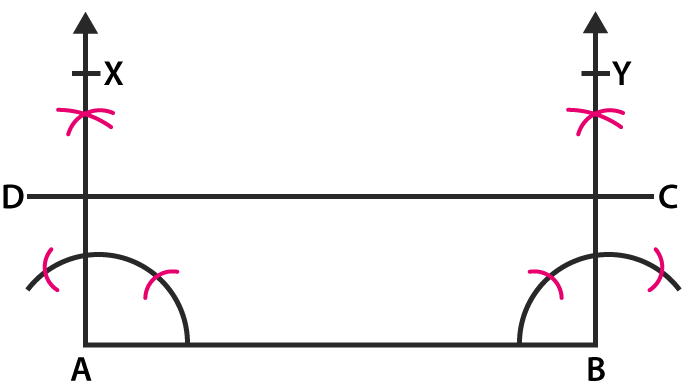# RD Sharma Solutions for Class 6 Maths Chapter 19: Geometrical Constructions

Students learn about simple and basic constructions in this Chapter using a ruler and a pair of compasses. The validity of measurements can be verified by using a ruler and protractor. This Chapter helps us understand the steps followed in various constructions like line segment, line to a given segment, line at a given point etc.

The solutions created by faculty are based on the latest syllabus to help students score well in the exam. Helping students to have a good academic score is the primary aim of preparing solutions. RD Sharma Solutions for Class 6 Chapter 19 Geometrical Constructions PDF are provided here.

## RD Sharma Solutions for Class 6 Maths Chapter 19: Geometrical Constructions Download PDF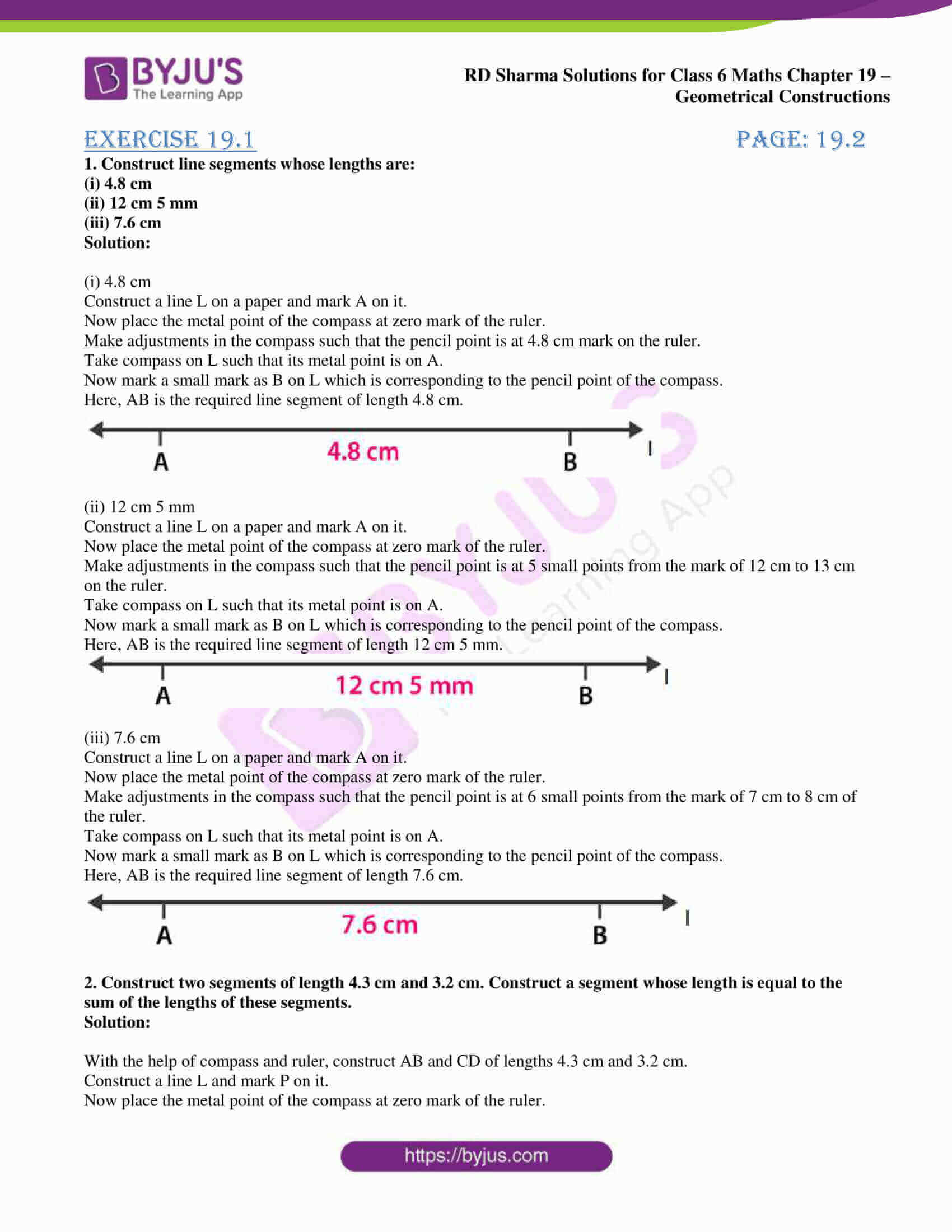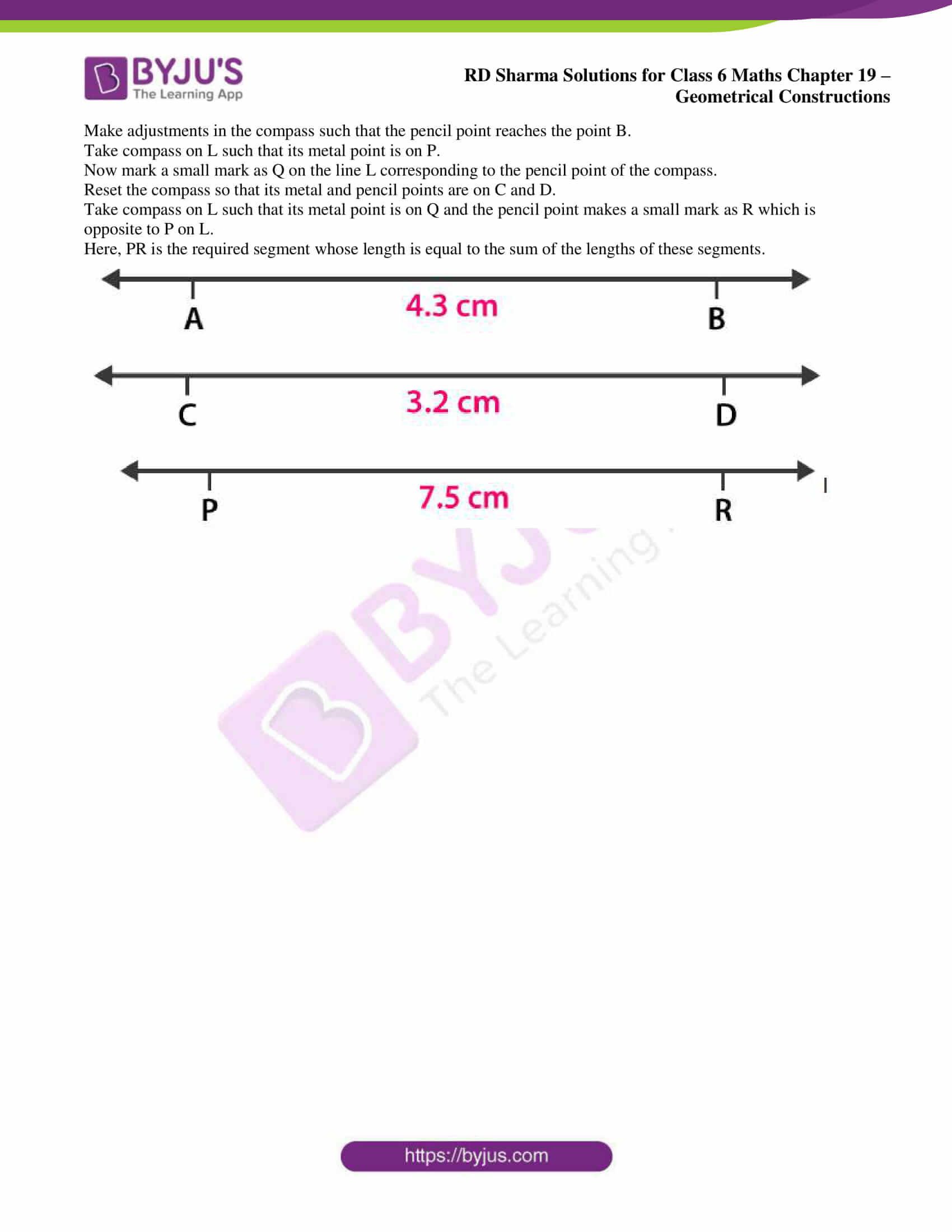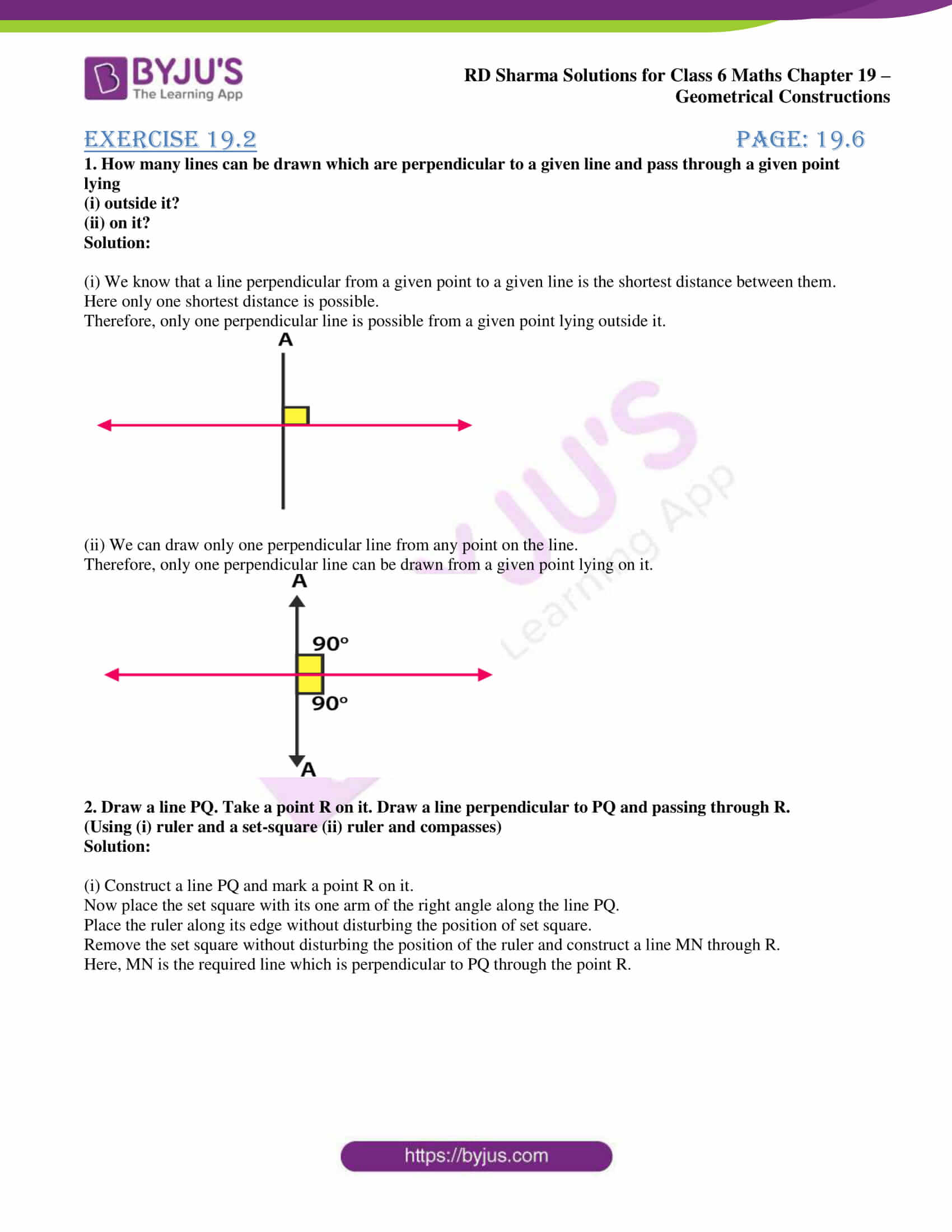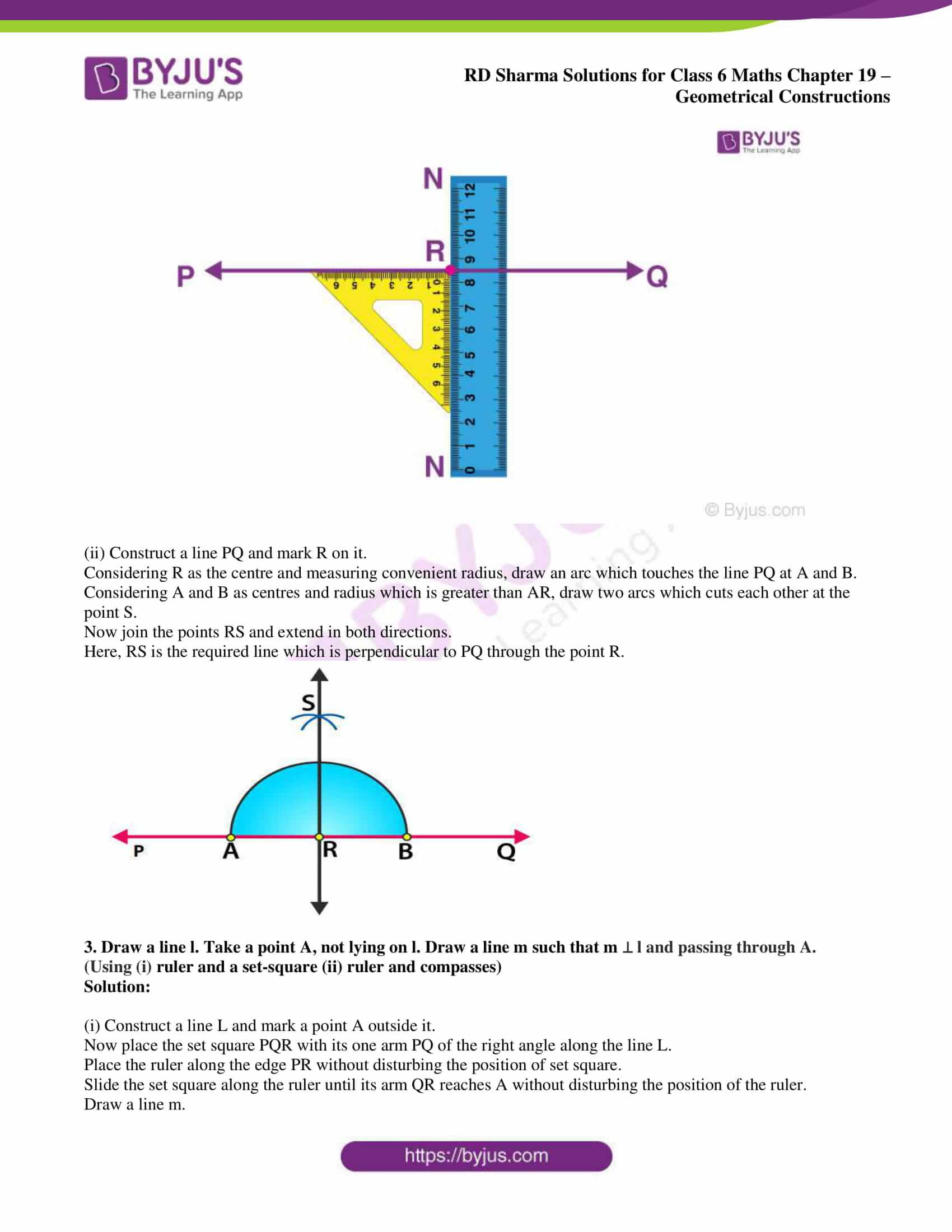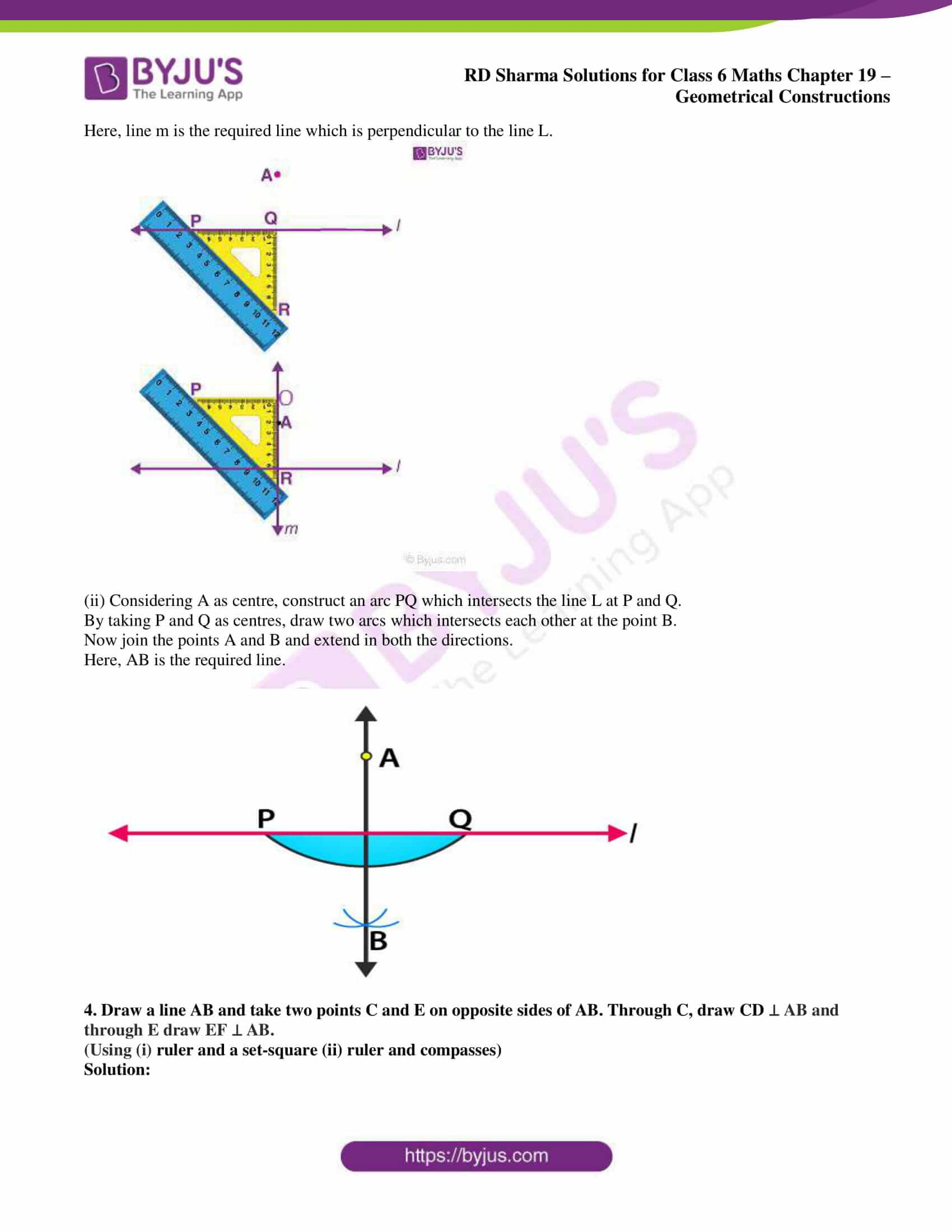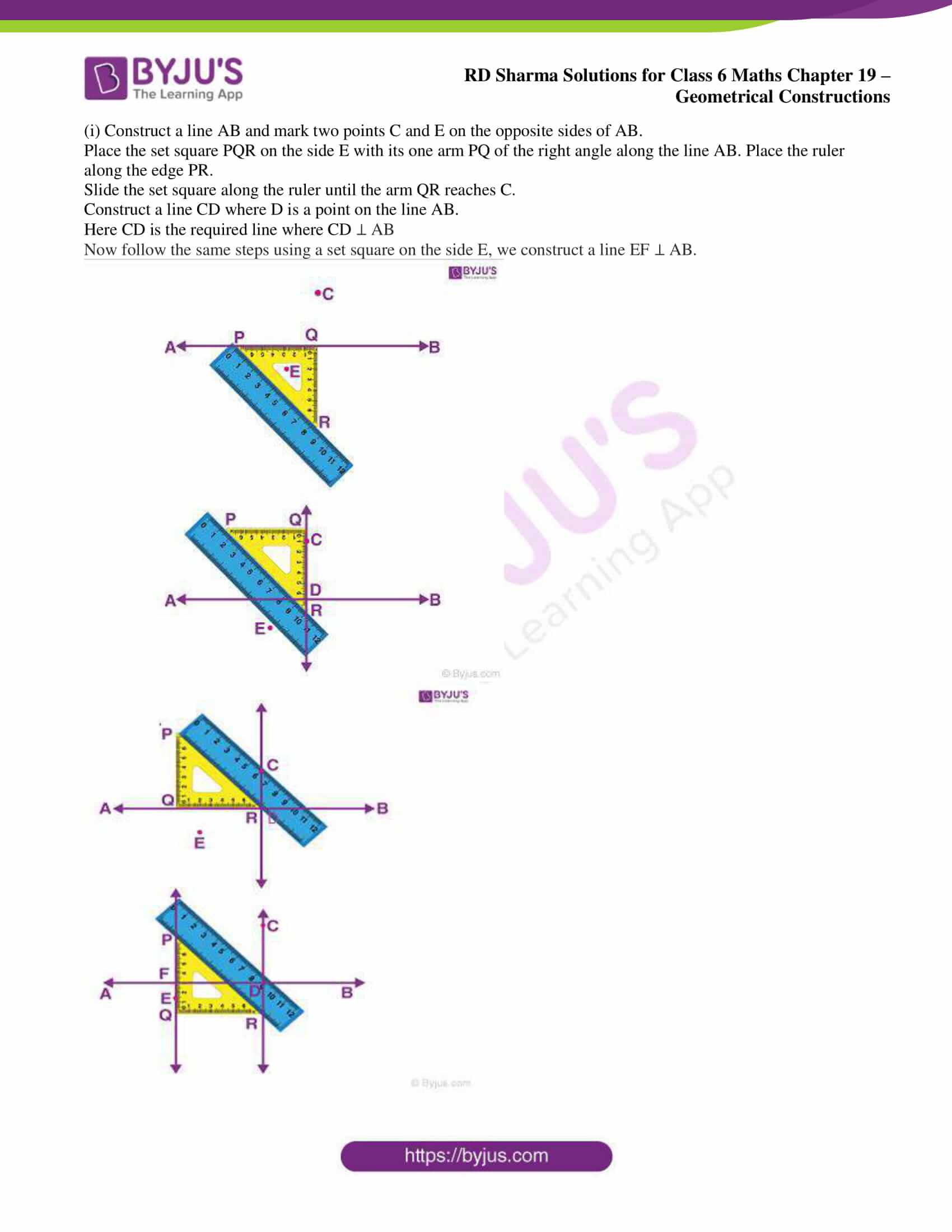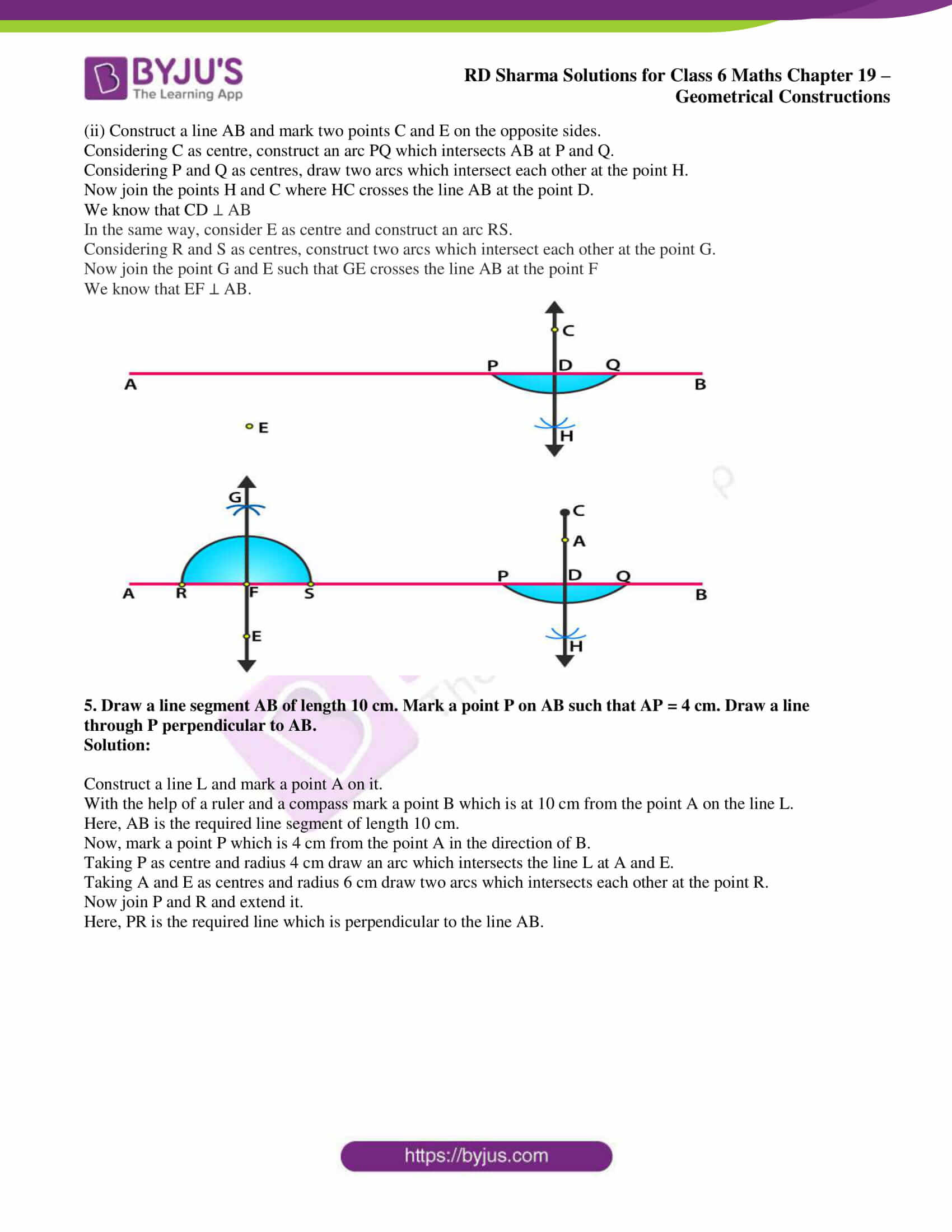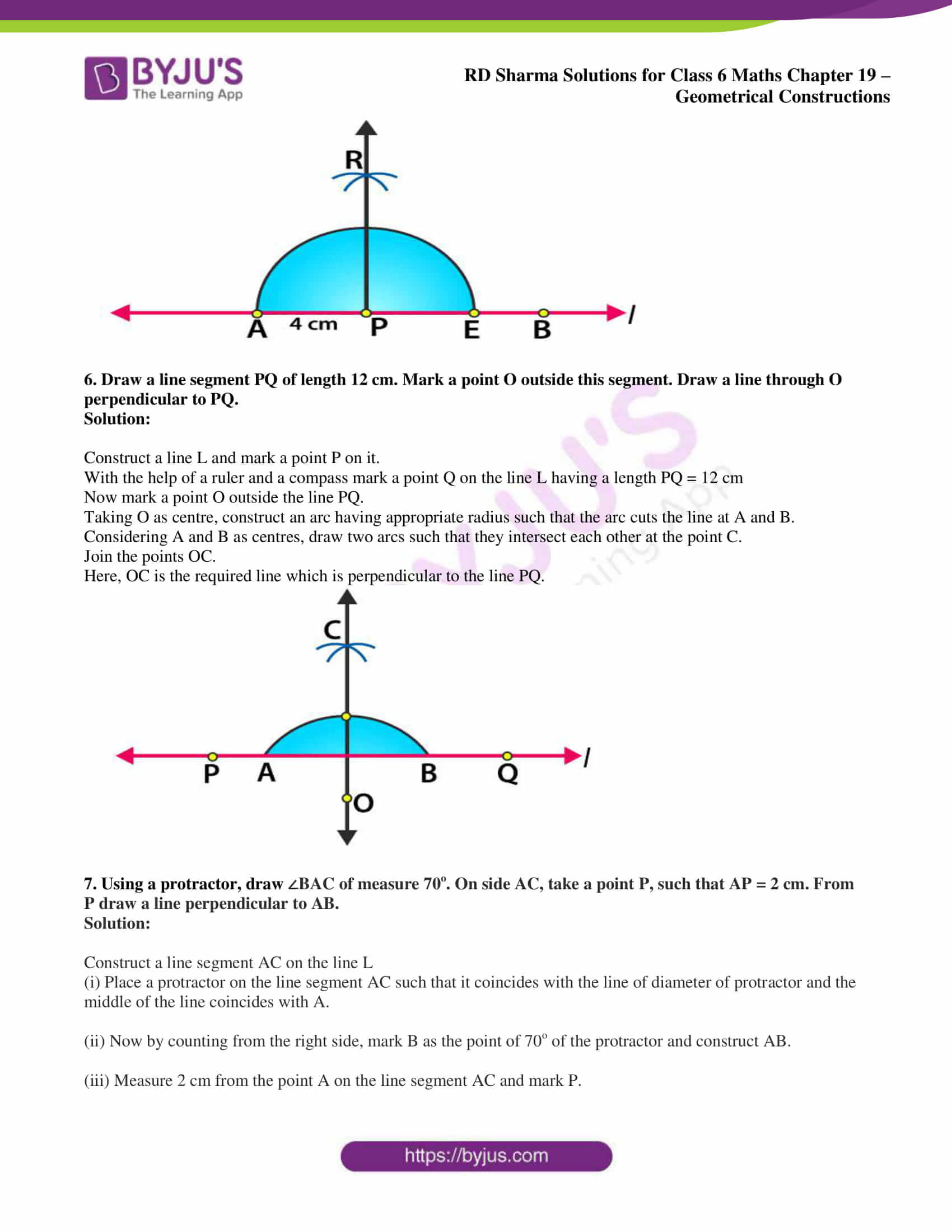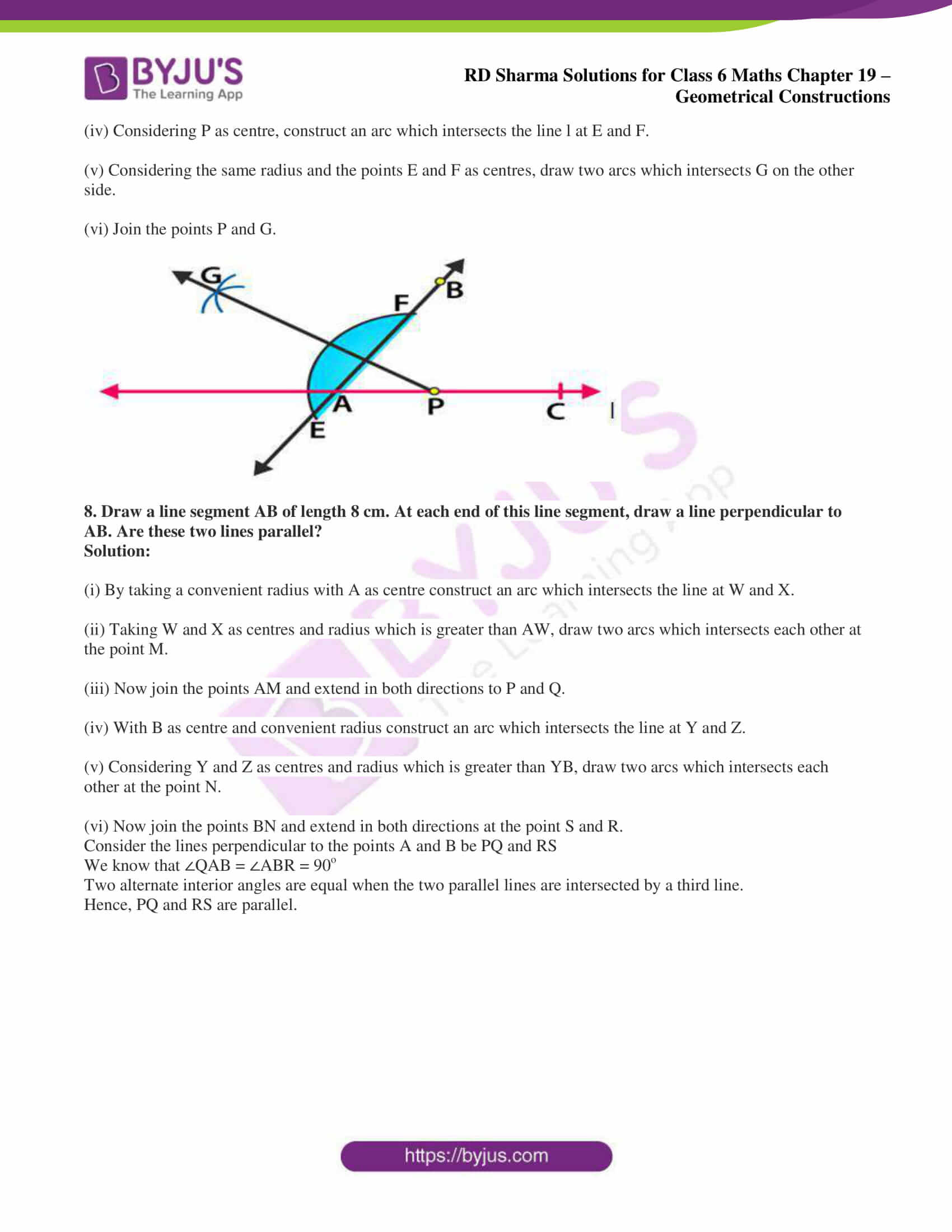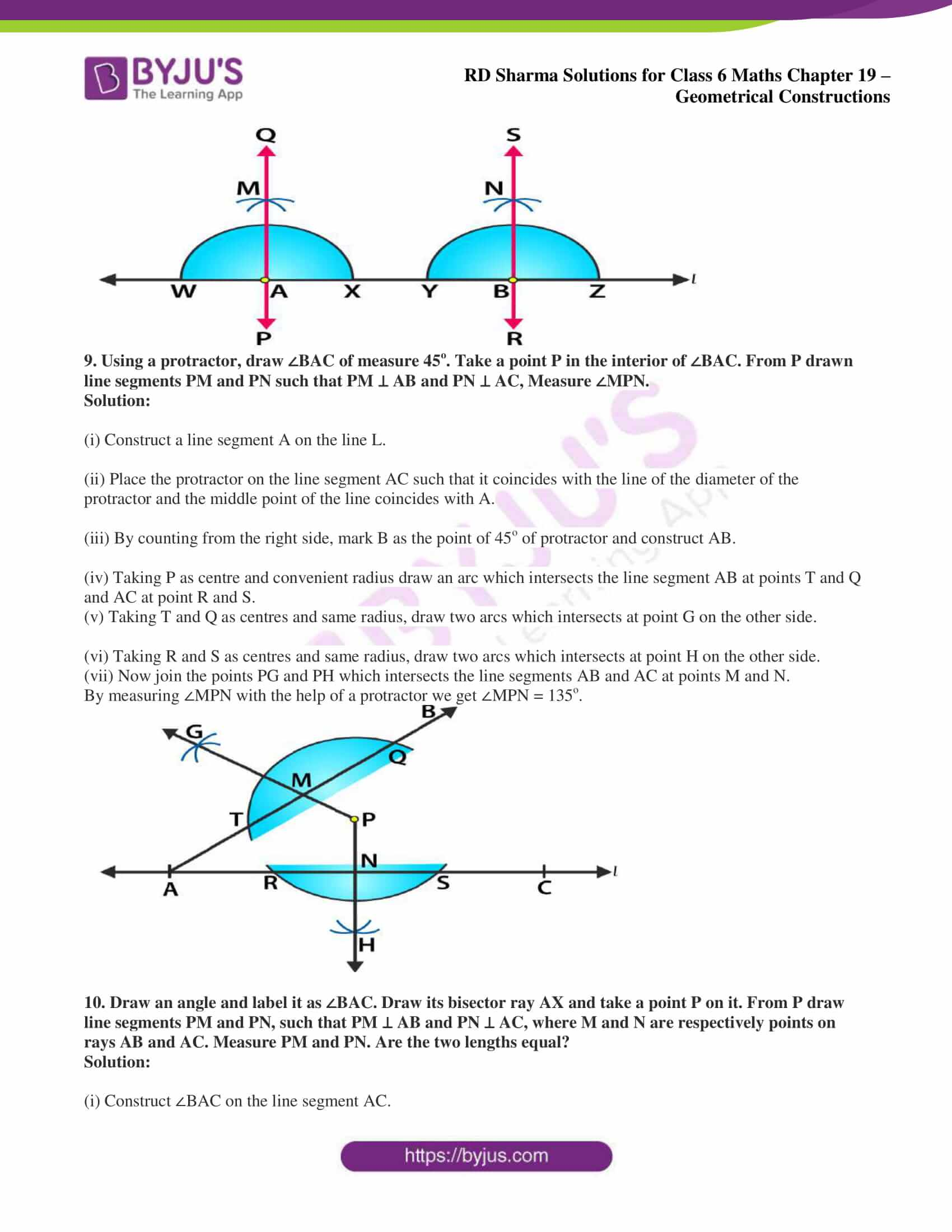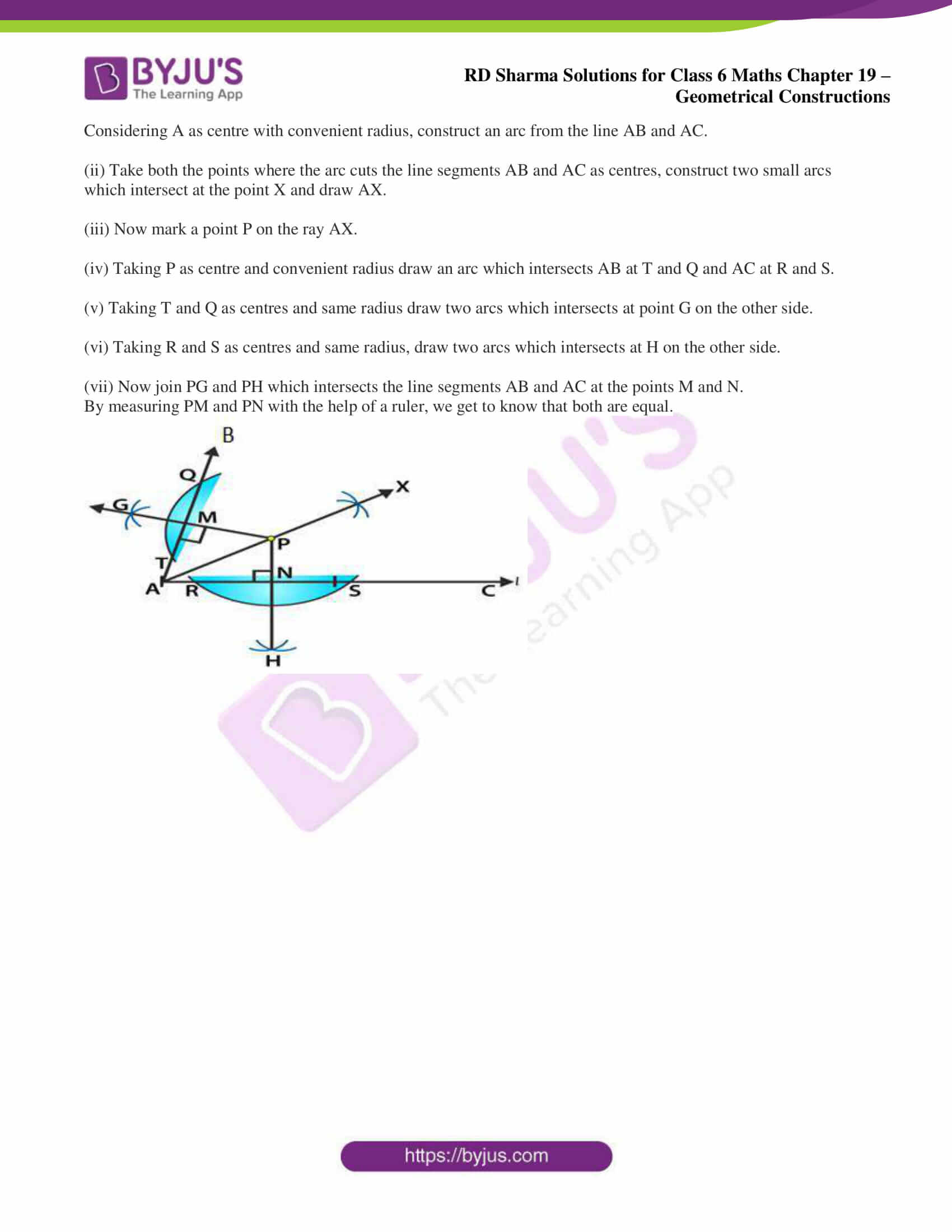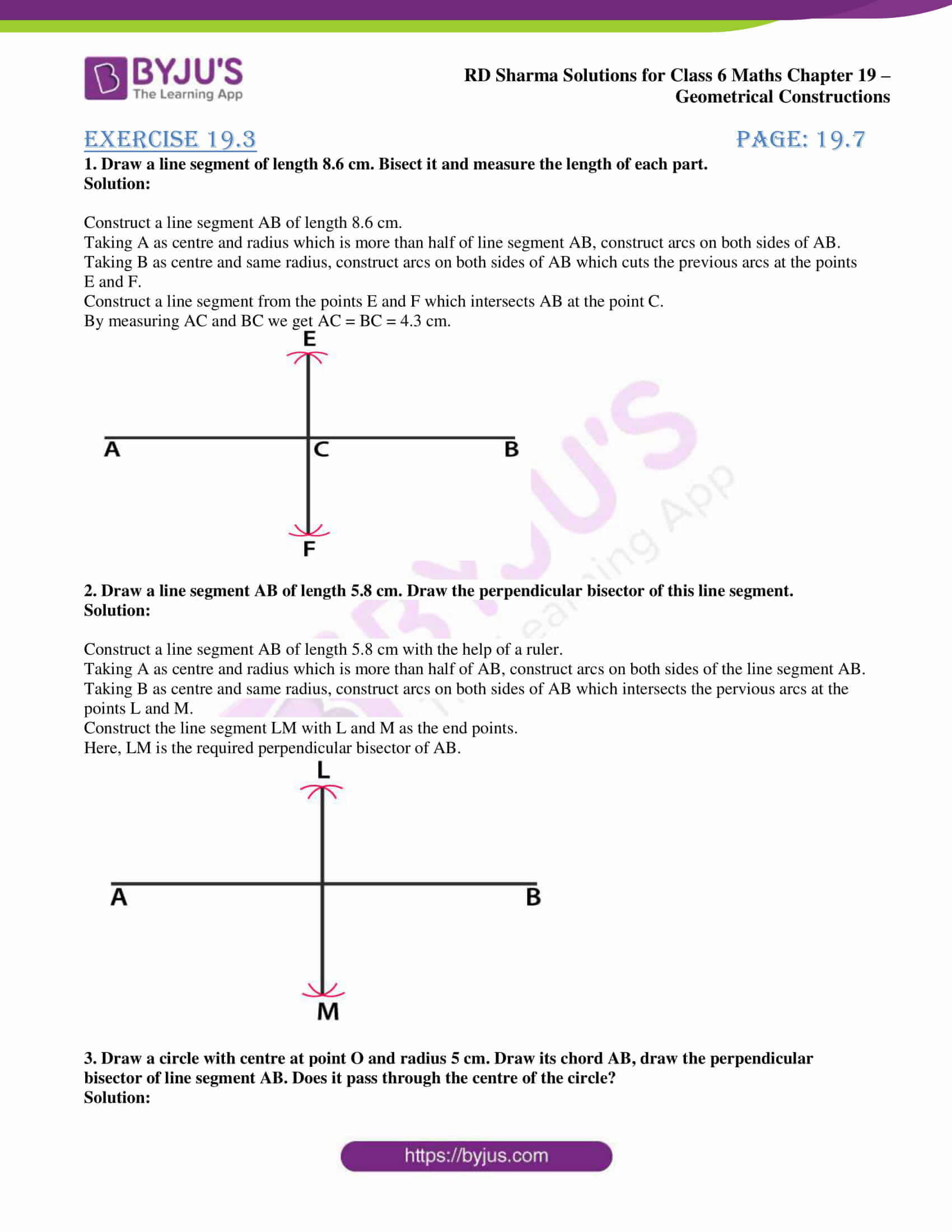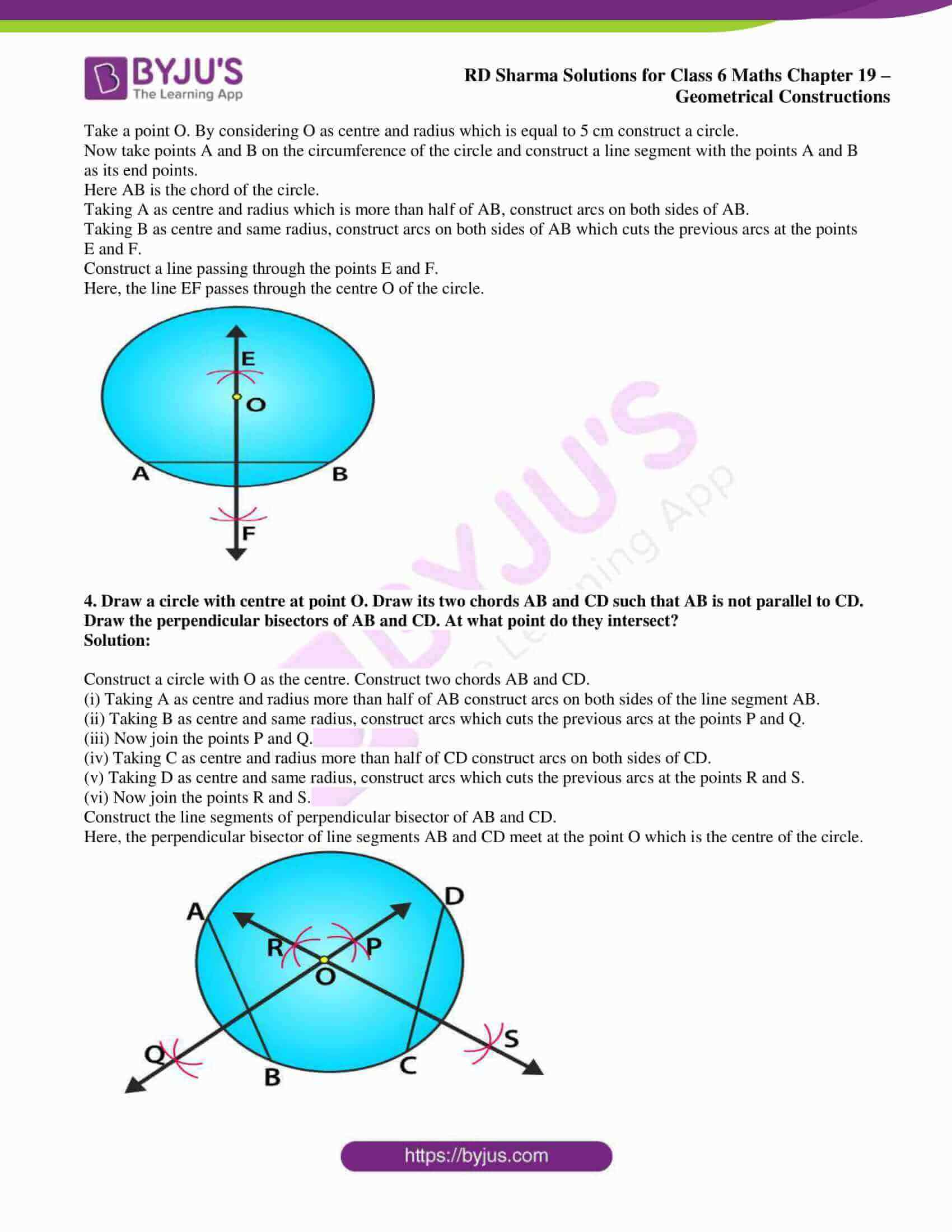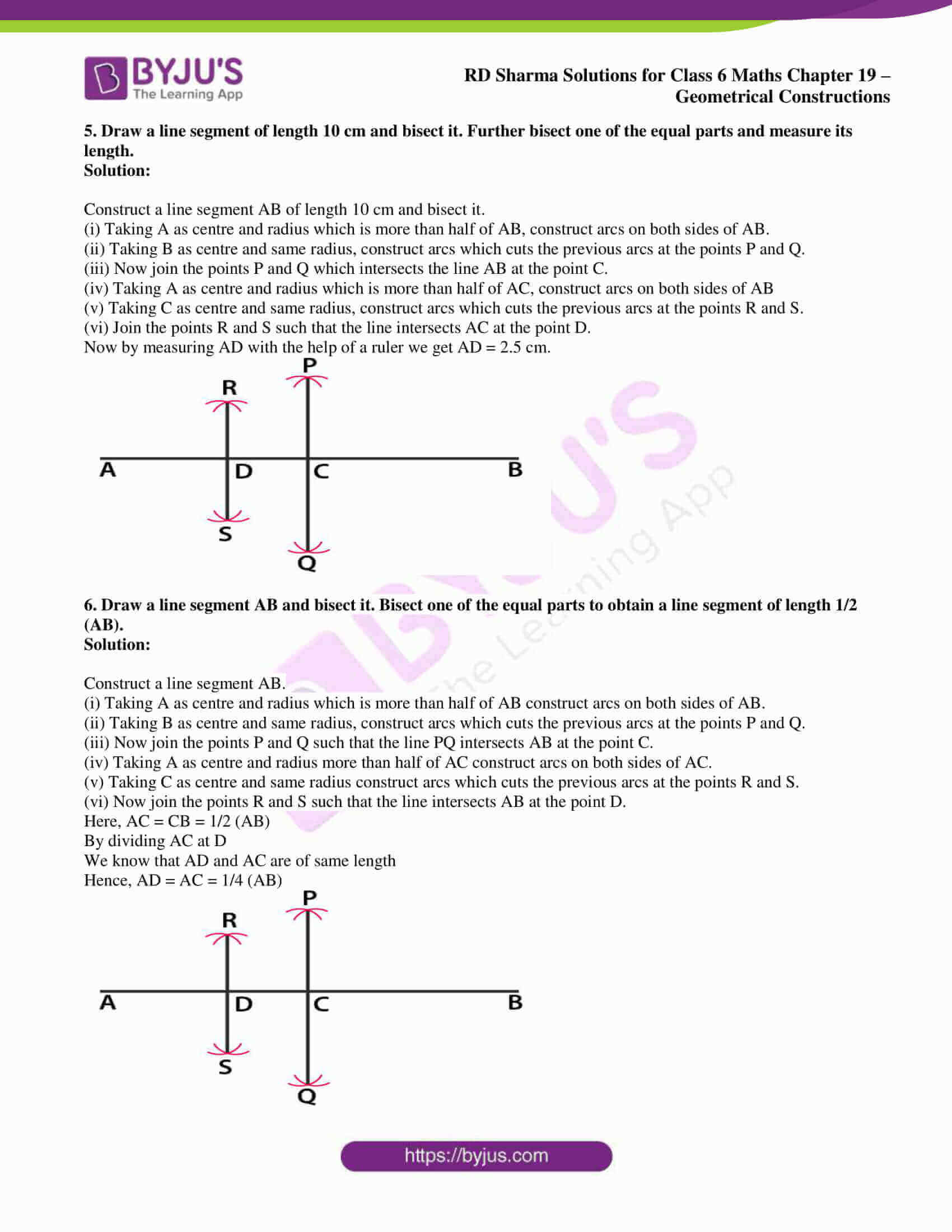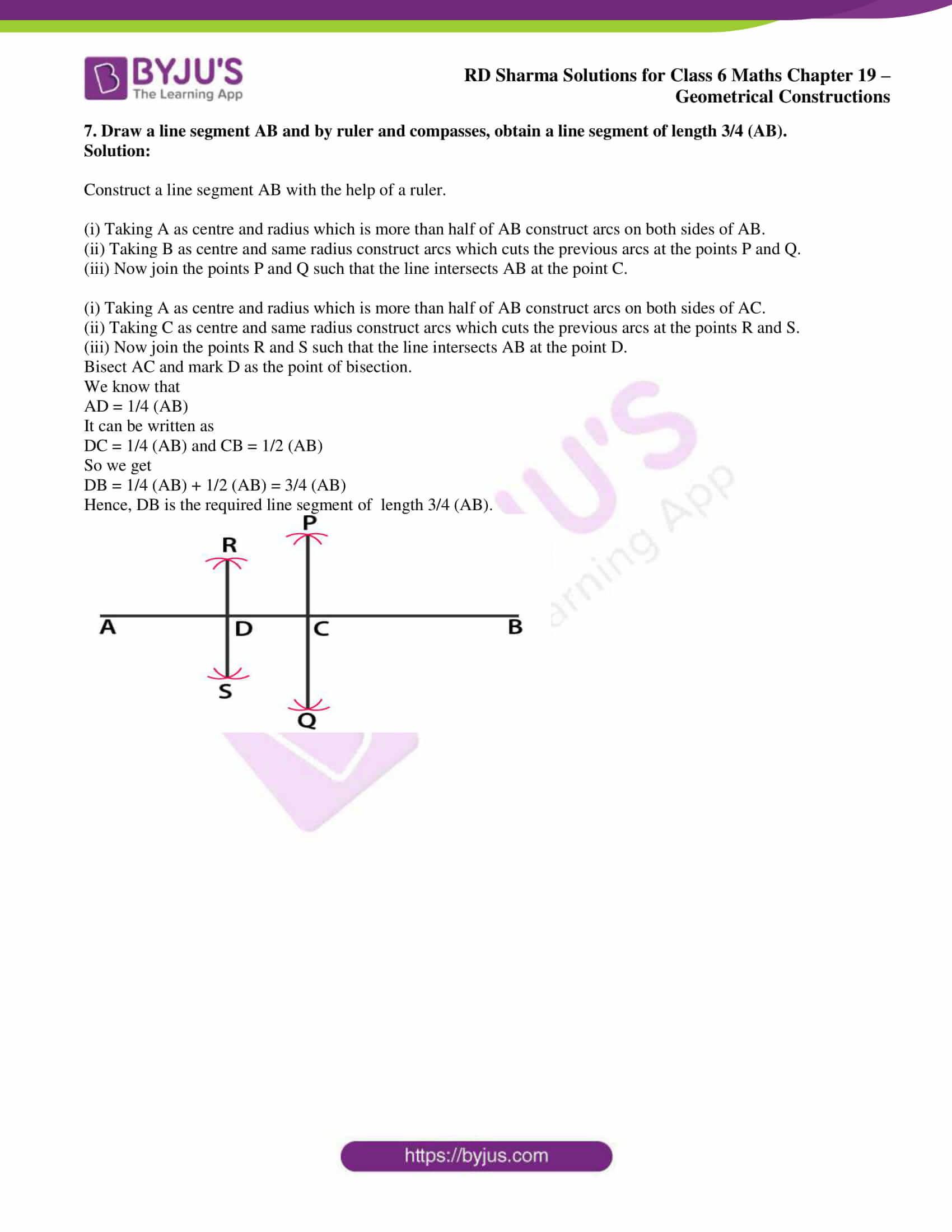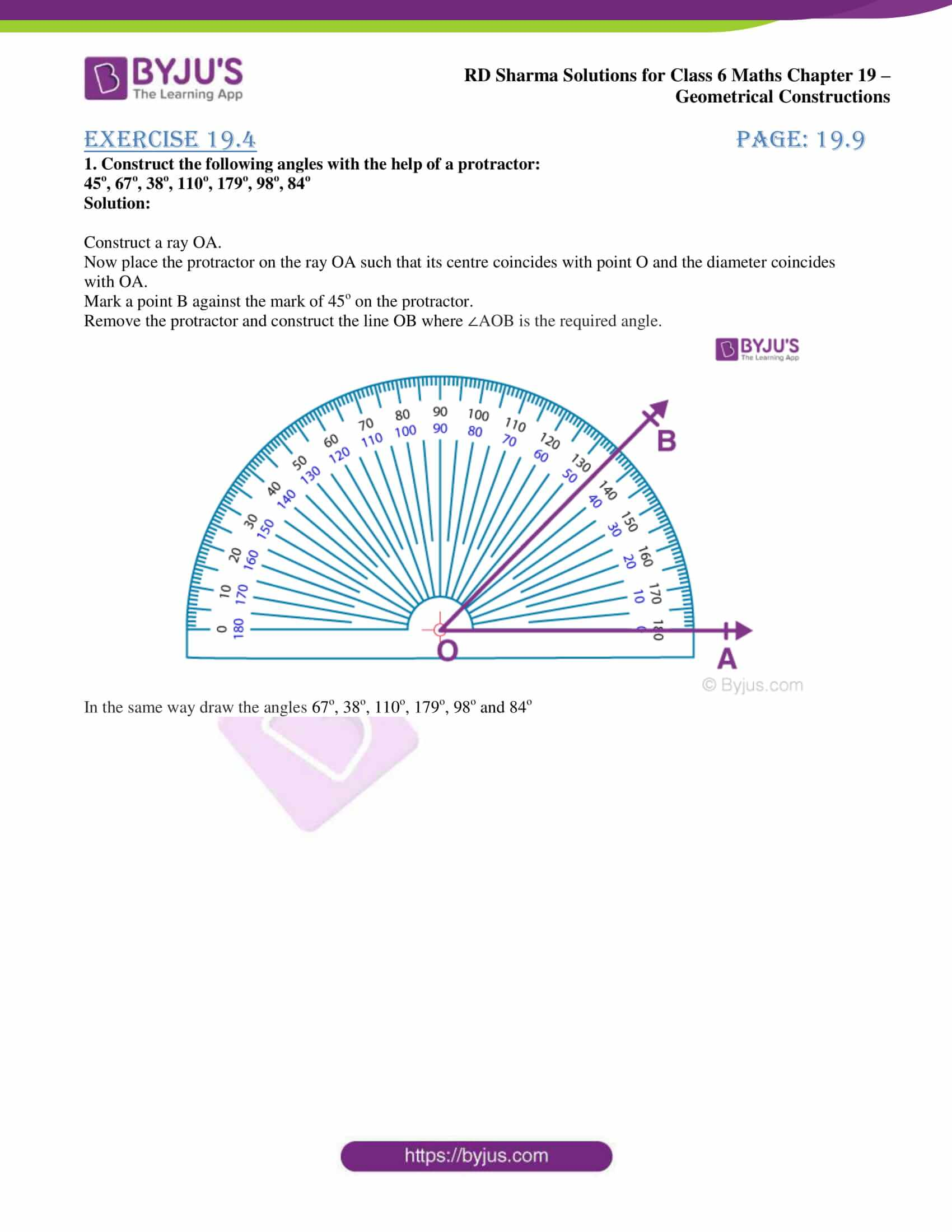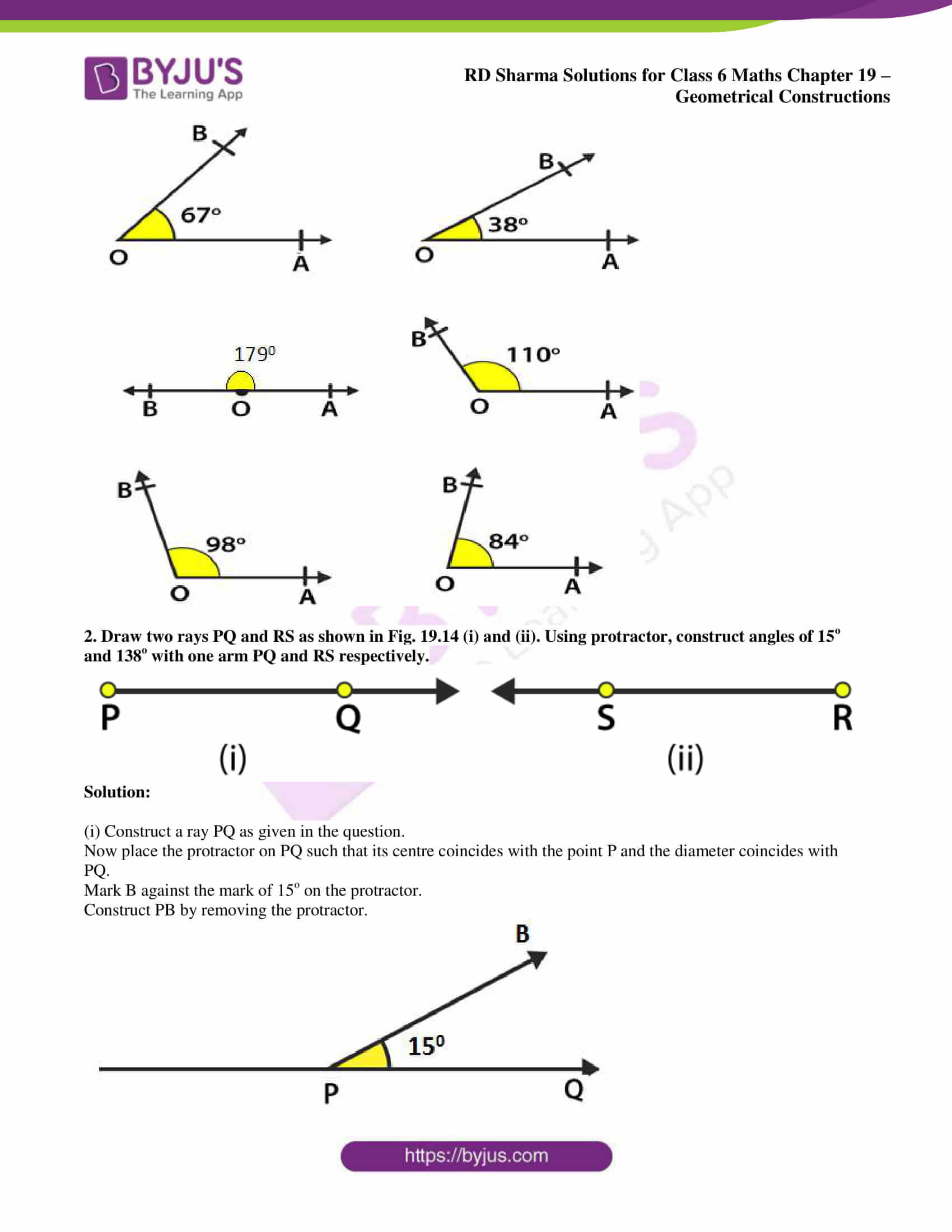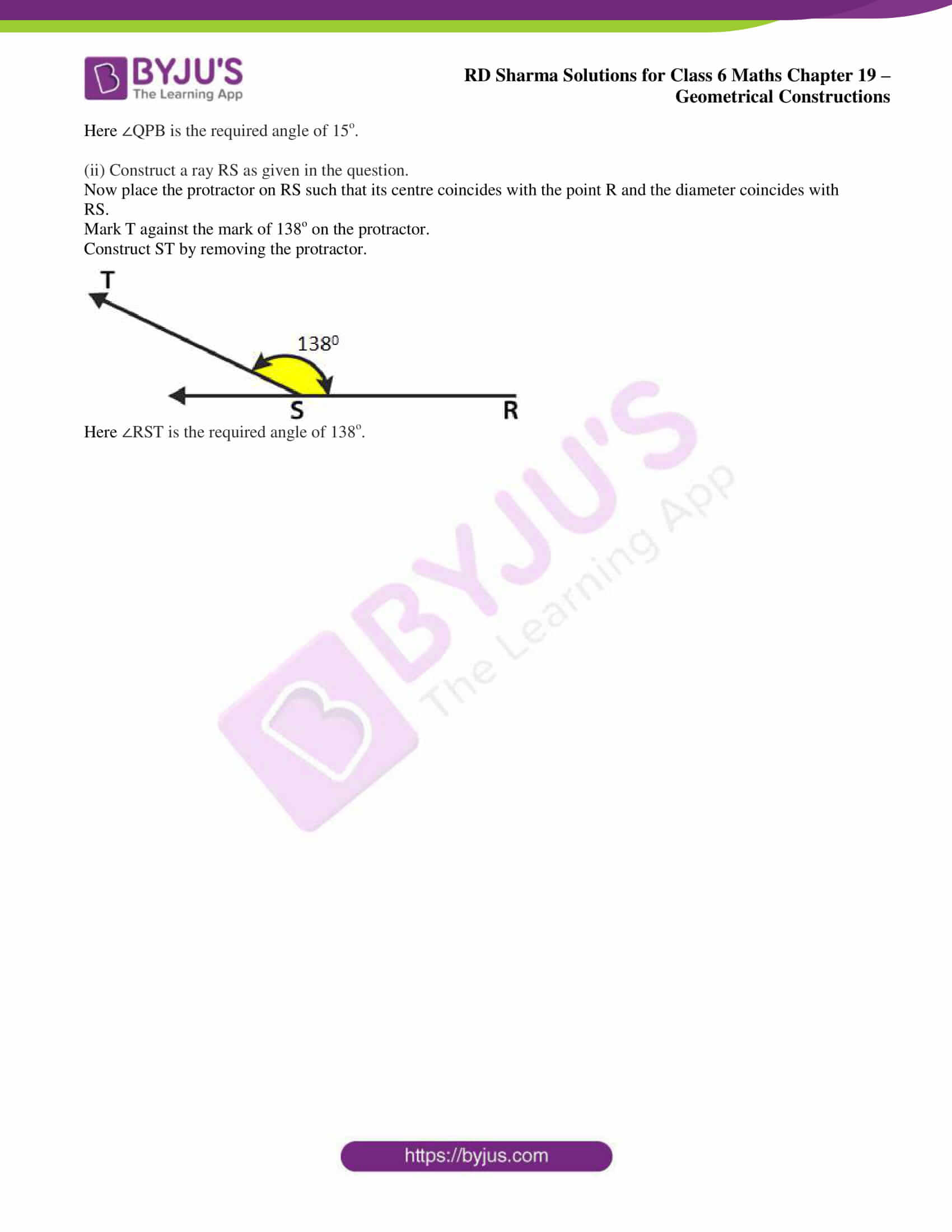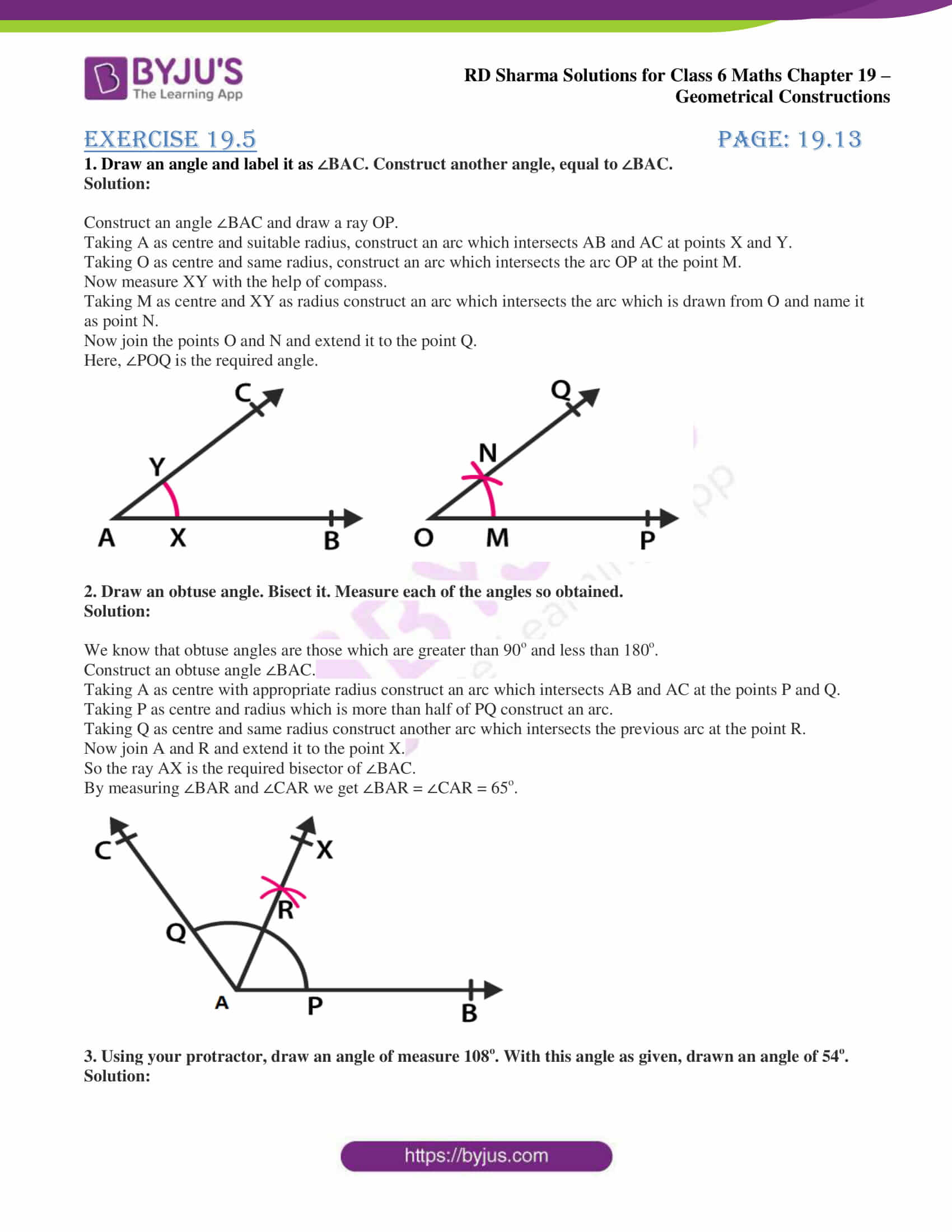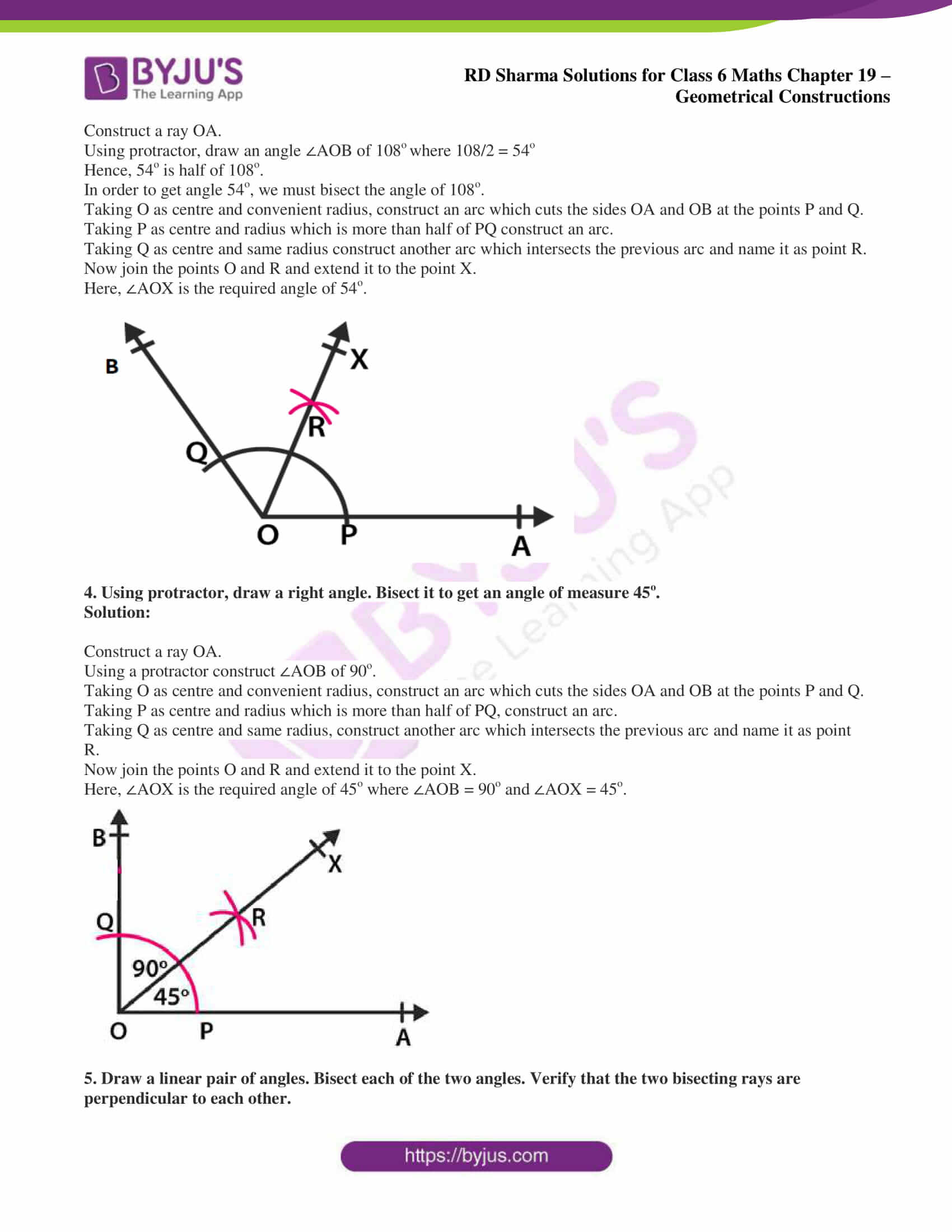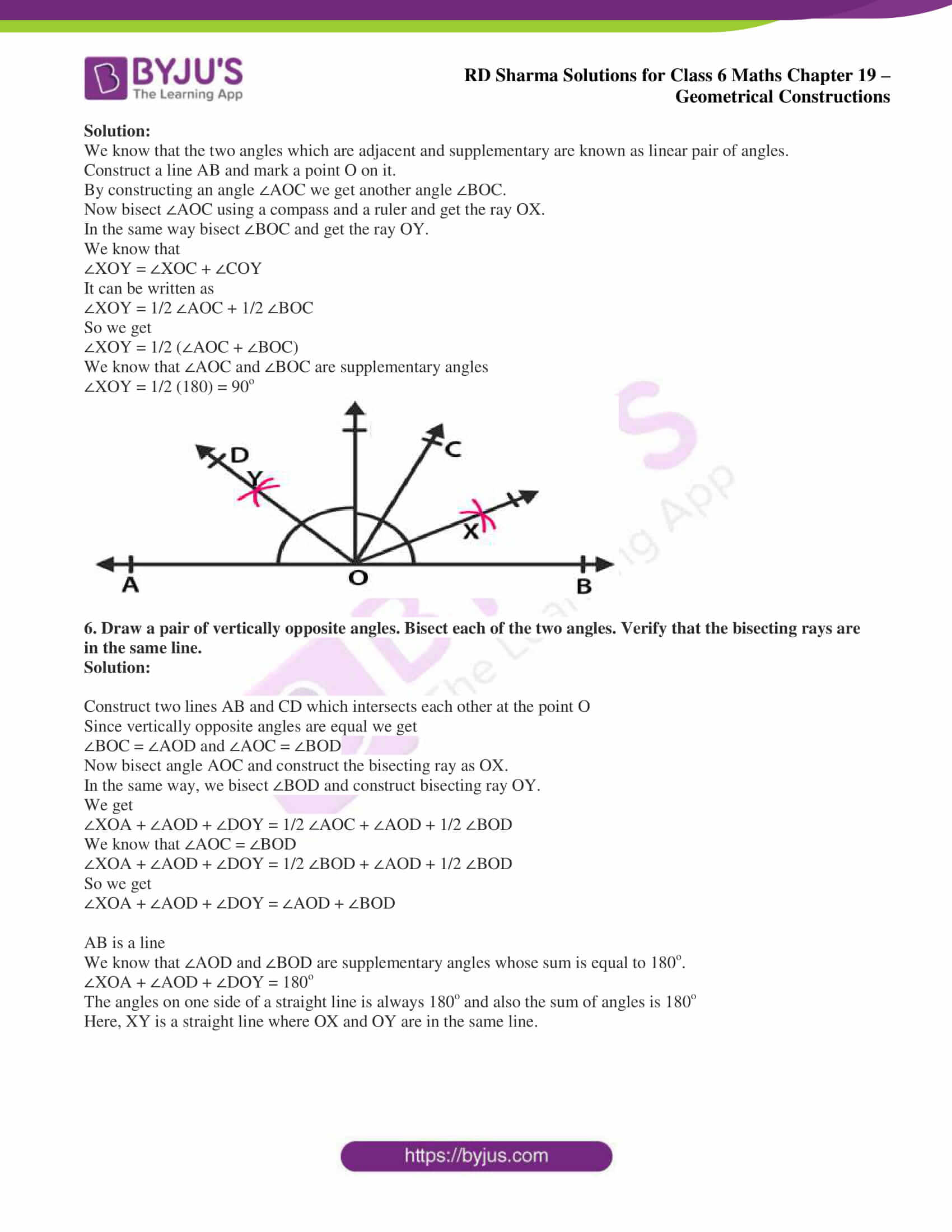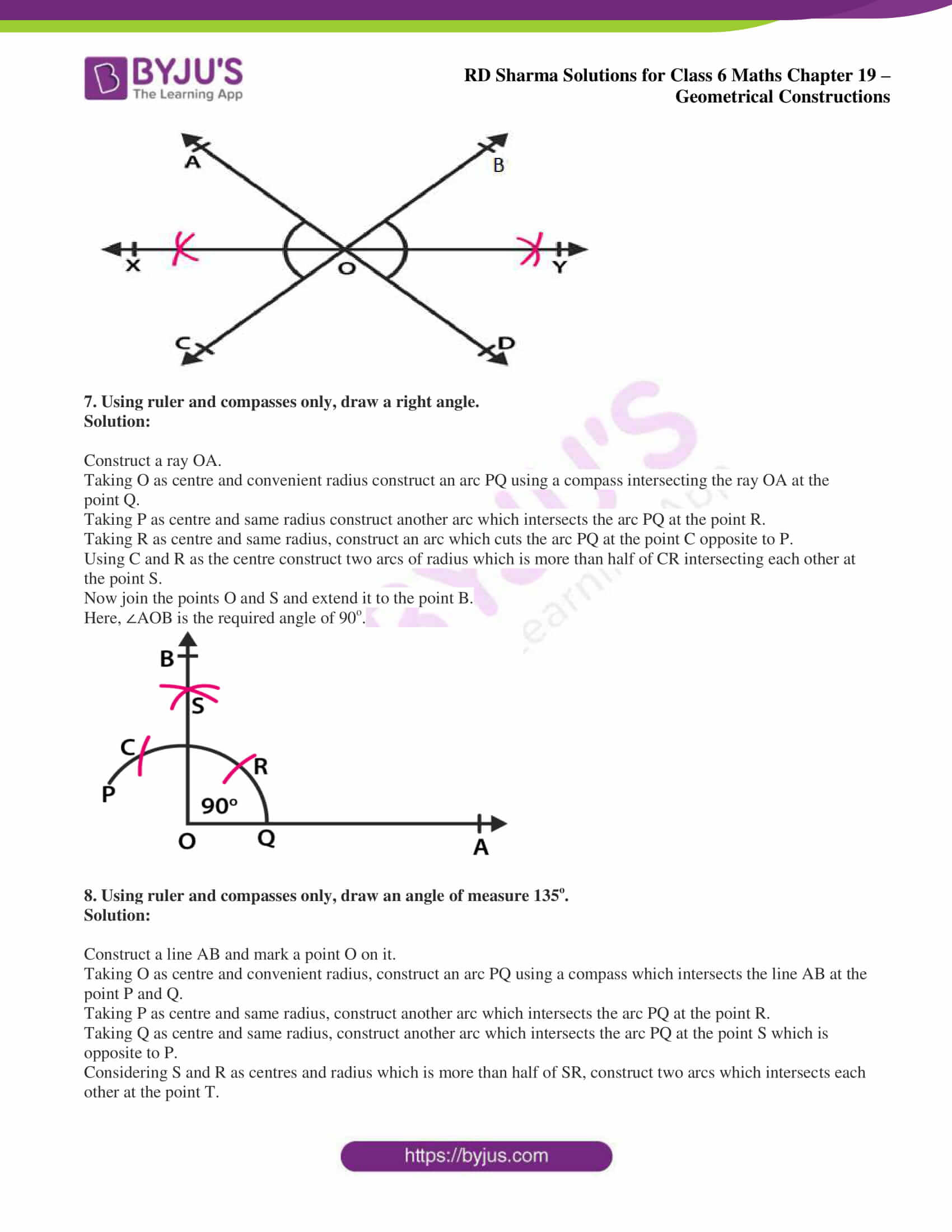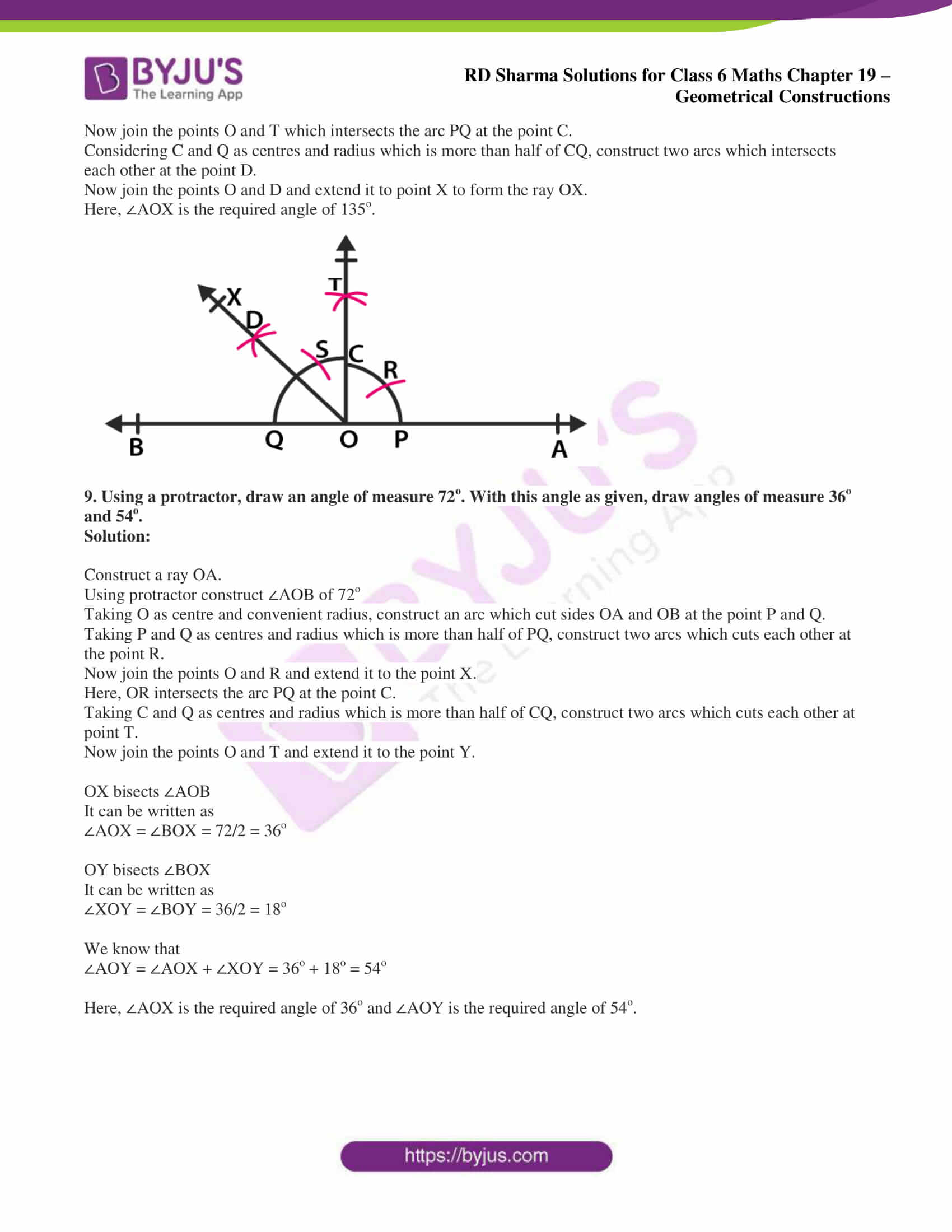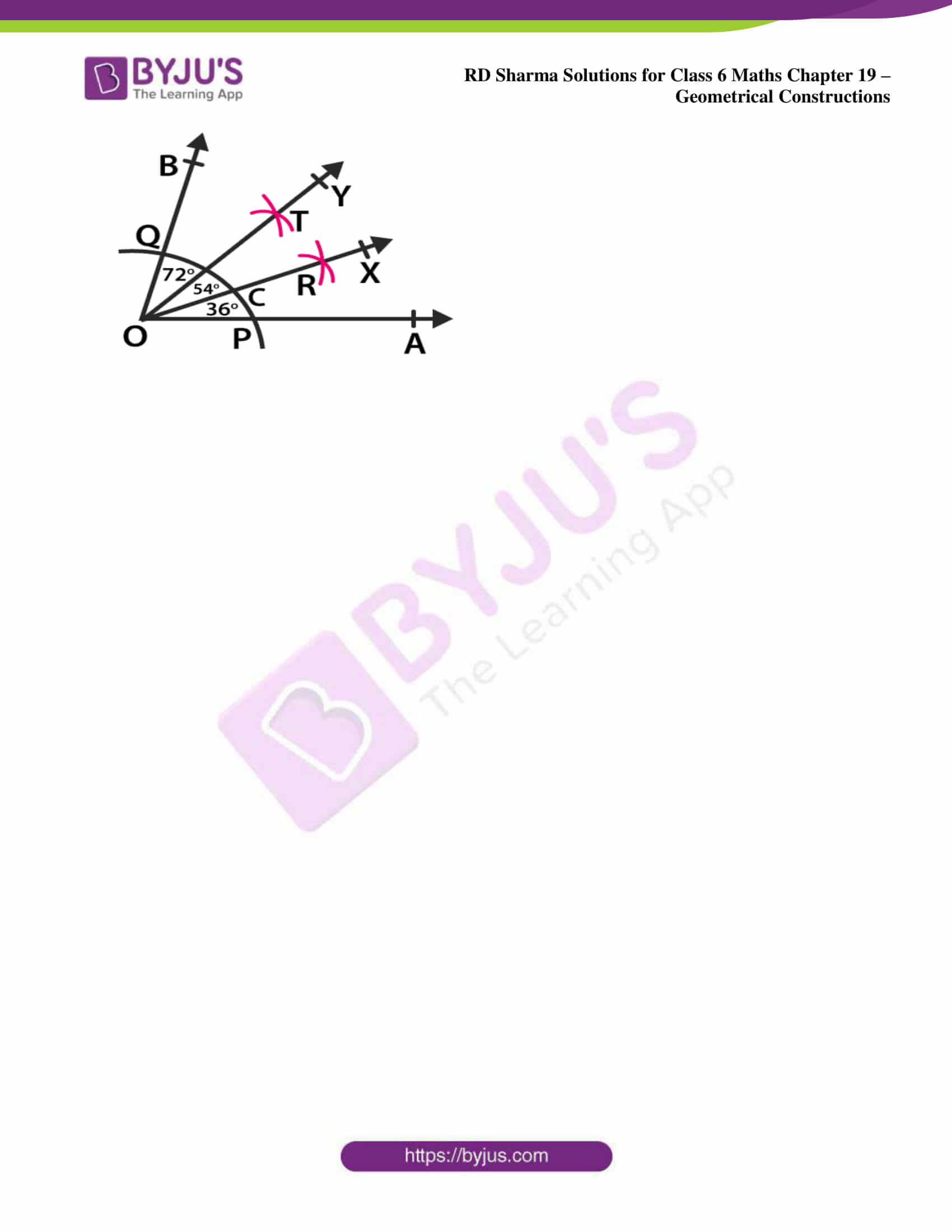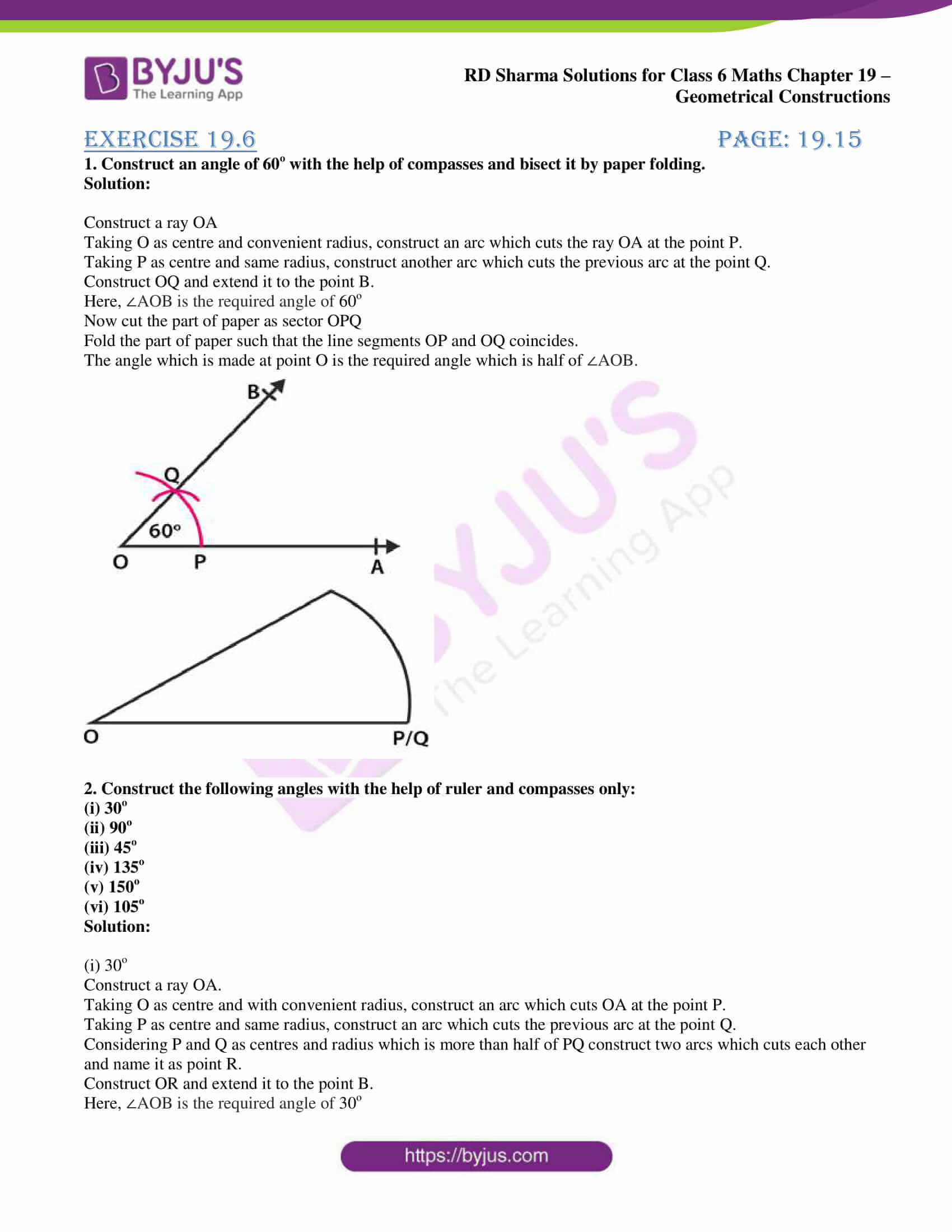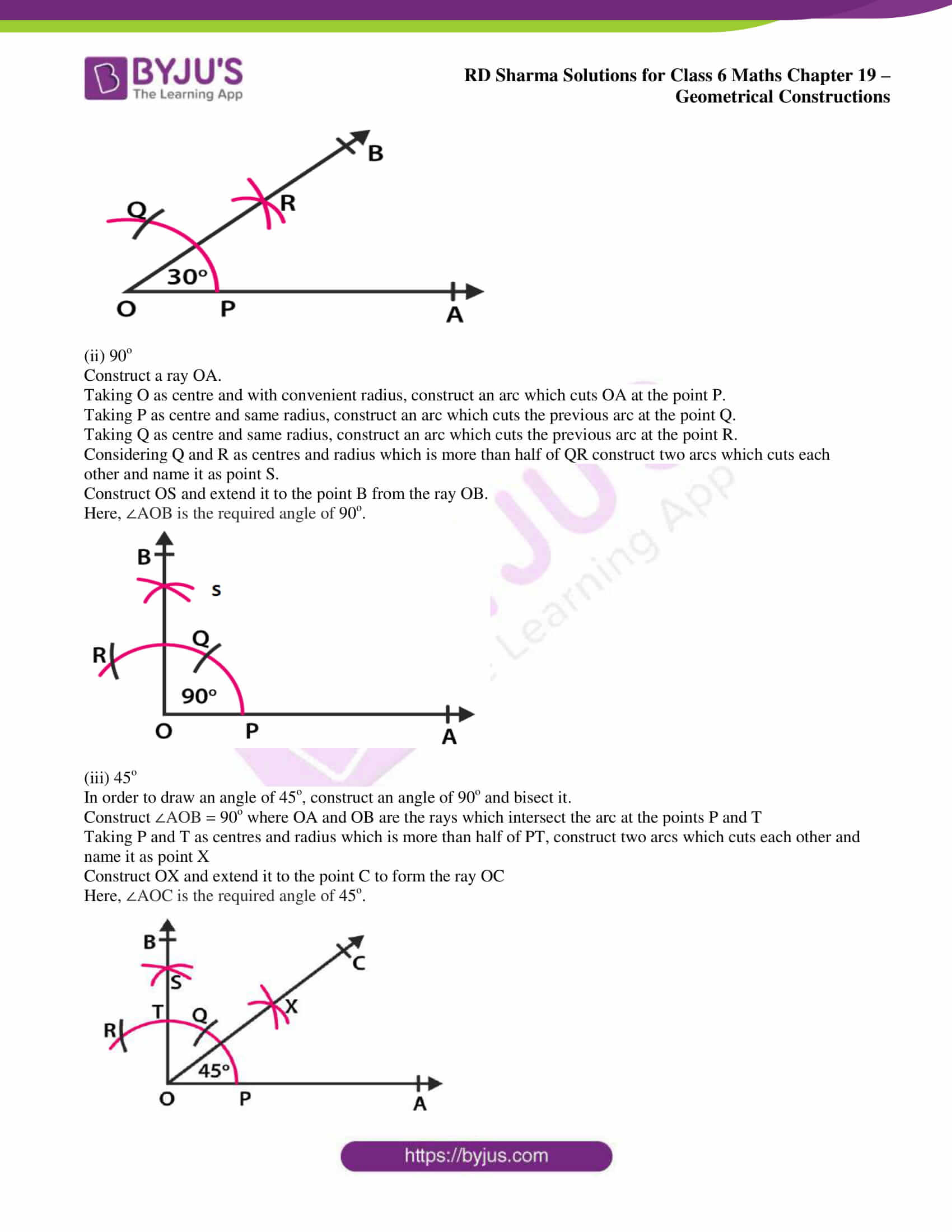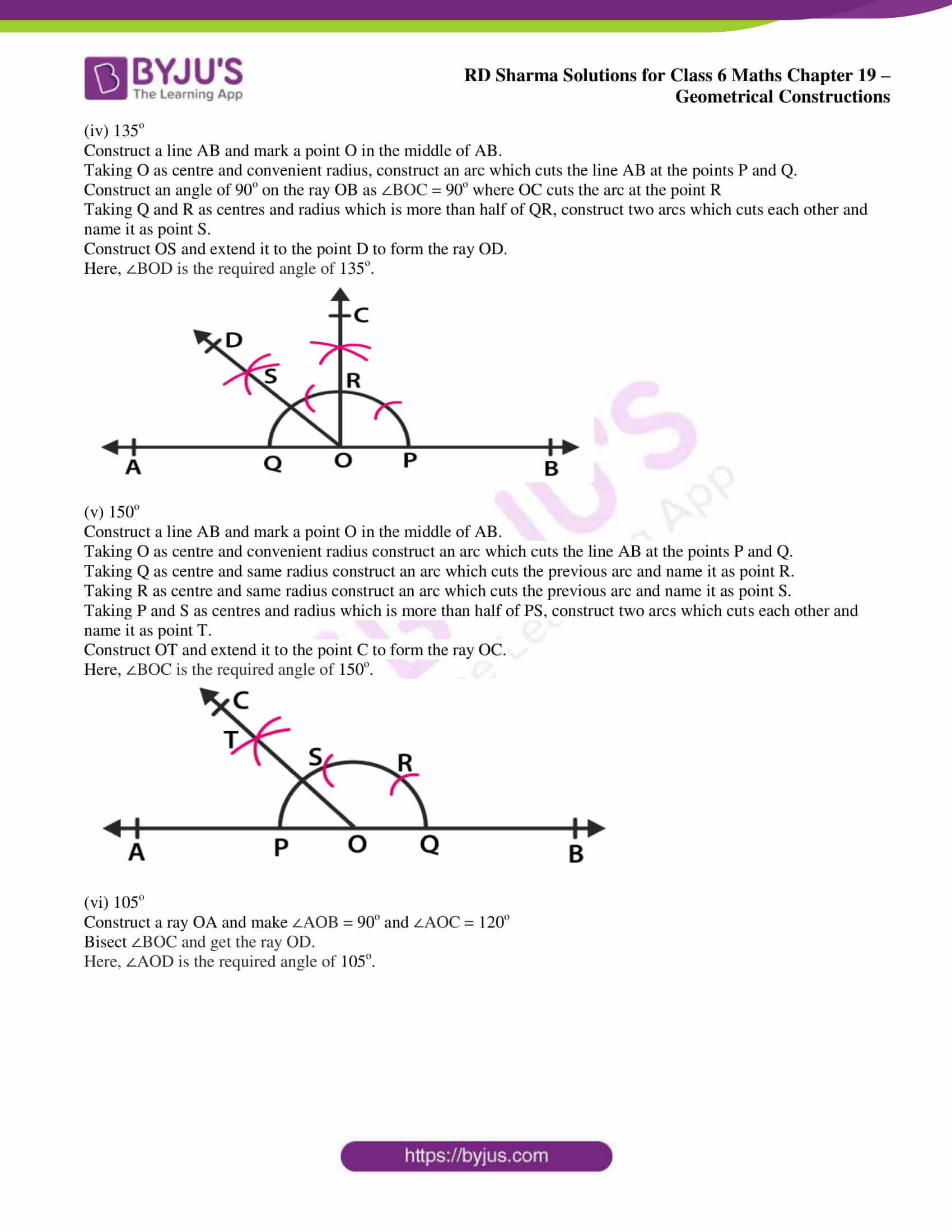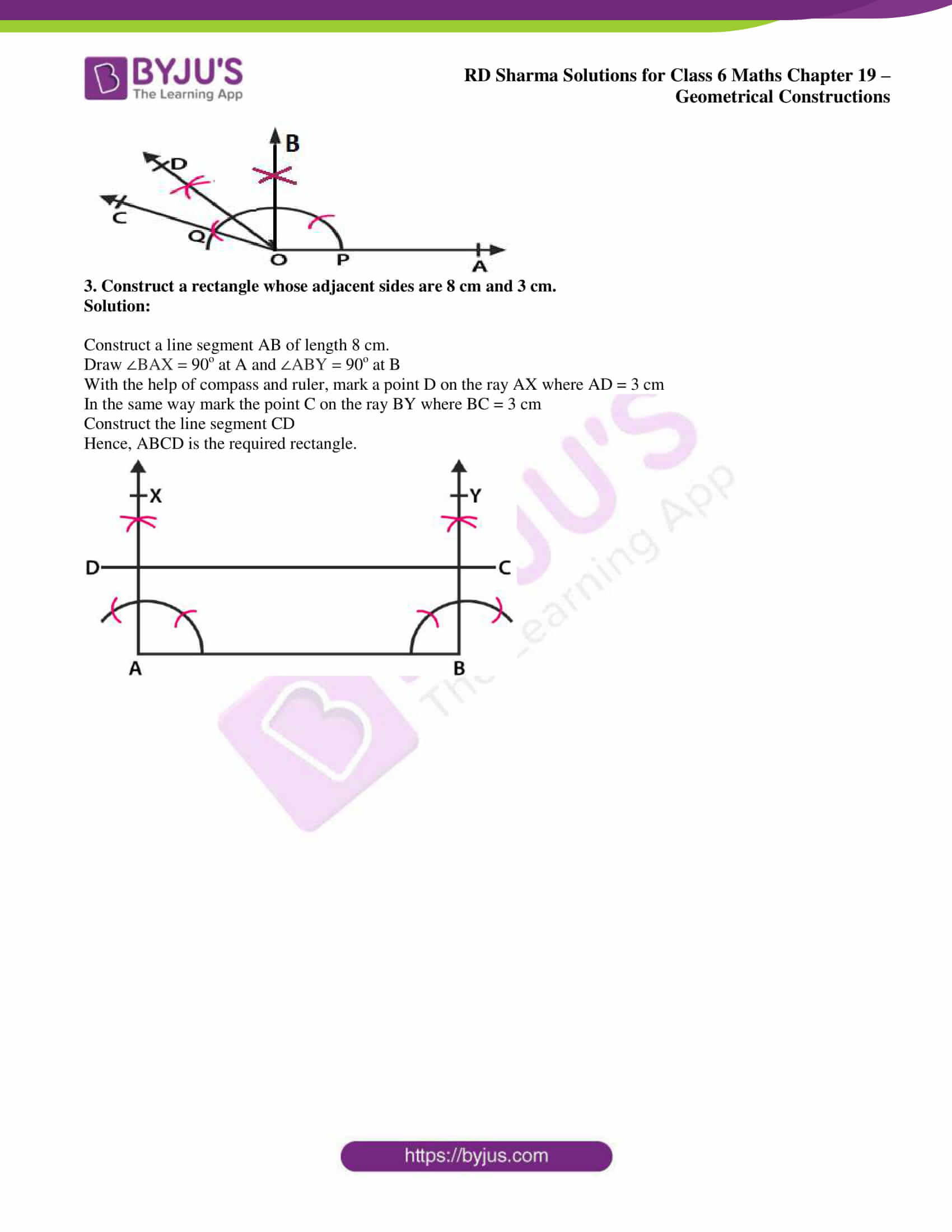## Access answers to Maths RD Sharma Solutions for Class 6 Chapter 19: Geometrical Constructions

### Exercise 19.1 page: 19.2

1. Construct line segments whose lengths are:

(i) 4.8 cm

(ii) 12 cm 5 mm

(iii) 7.6 cm

Solution:

(i) 4.8 cm

Construct a line L on a paper and mark A on it.

Now place the metal point of the compass at zero mark of the ruler.

Make adjustments in the compass such that the pencil point is at 4.8 cm mark on the ruler.

Take compass on L such that its metal point is on A.

Now mark a small mark as B on L which is corresponding to the pencil point of the compass.

Here, AB is the required line segment of length 4.8 cm.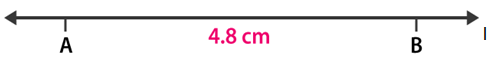(ii) 12 cm 5 mm

Construct a line L on a paper and mark A on it.

Now place the metal point of the compass at zero mark of the ruler.

Make adjustments in the compass such that the pencil point is at 5 small points from the mark of 12 cm to 13 cm on the ruler.

Take compass on L such that its metal point is on A.

Now mark a small mark as B on L which is corresponding to the pencil point of the compass.

Here, AB is the required line segment of length 12 cm 5 mm.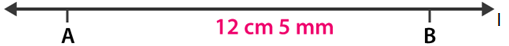(iii) 7.6 cm

Construct a line L on a paper and mark A on it.

Now place the metal point of the compass at zero mark of the ruler.

Make adjustments in the compass such that the pencil point is at 6 small points from the mark of 7 cm to 8 cm of the ruler.

Take compass on L such that its metal point is on A.

Now mark a small mark as B on L which is corresponding to the pencil point of the compass.

Here, AB is the required line segment of length 7.6 cm.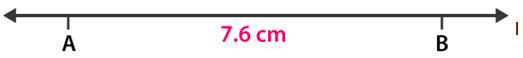2. Construct two segments of length 4.3 cm and 3.2 cm. Construct a segment whose length is equal to the sum of the lengths of these segments.

Solution:

With the help of compass and ruler, construct AB and CD of lengths 4.3 cm and 3.2 cm.

Construct a line L and mark P on it.

Now place the metal point of the compass at zero mark of the ruler.

Make adjustments in the compass such that the pencil point reaches the point B.

Take compass on L such that its metal point is on P.

Now mark a small mark as Q on the line L corresponding to the pencil point of the compass.

Reset the compass so that its metal and pencil points are on C and D.

Take compass on L such that its metal point is on Q and the pencil point makes a small mark as R which is opposite to P on L.

Here, PR is the required segment whose length is equal to the sum of the lengths of these segments.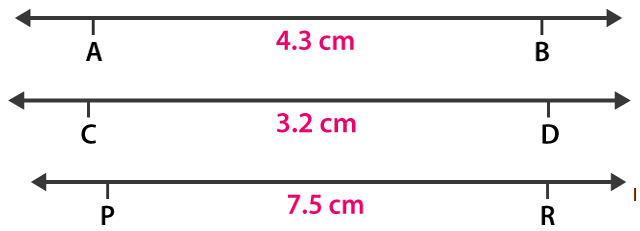### Exercise 19.2 page: 19.6

1. How many lines can be drawn which are perpendicular to a given line and pass through a given point lying

(i) outside it?

(ii) on it?

Solution:

(i) We know that a line perpendicular from a given point to a given line is the shortest distance between them.

Here only one shortest distance is possible.

Therefore, only one perpendicular line is possible from a given point lying outside it.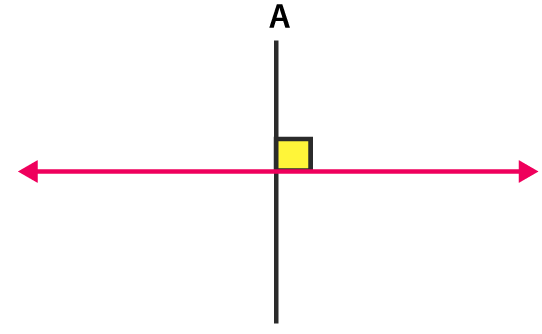(ii) We can draw only one perpendicular line from any point on the line.

Therefore, only one perpendicular line can be drawn from a given point lying on it.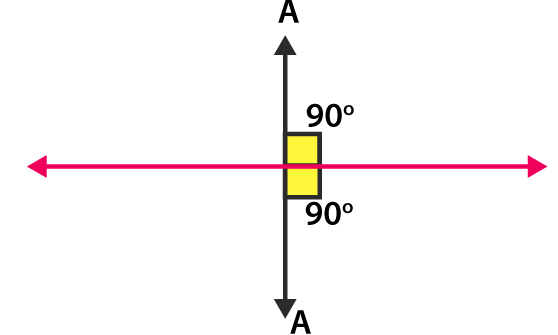2. Draw a line PQ. Take a point R on it. Draw a line perpendicular to PQ and passing through R.

(Using (i) ruler and a set-square (ii) ruler and compasses)

Solution:

(i) Construct a line PQ and mark a point R on it.

Now place the set square with its one arm of the right angle along the line PQ.

Place the ruler along its edge without disturbing the position of set square.

Remove the set square without disturbing the position of the ruler and construct a line MN through R.

Here, MN is the required line which is perpendicular to PQ through the point R.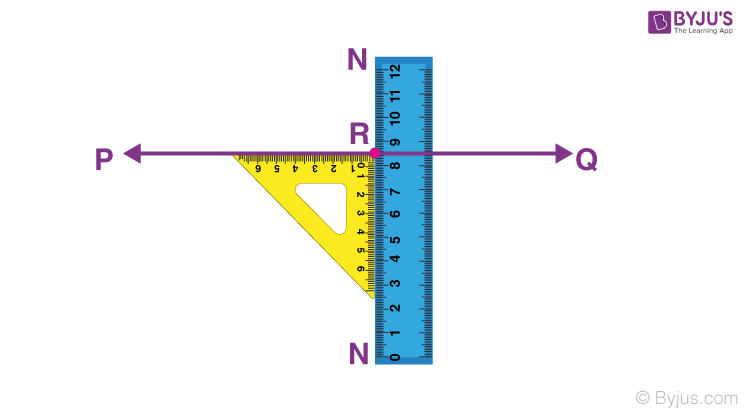(ii) Construct a line PQ and mark R on it.

Considering R as the centre and measuring convenient radius, draw an arc which touches the line PQ at A and B.

Considering A and B as centres and radius which is greater than AR, draw two arcs which cuts each other at the point S.

Now join the points RS and extend in both directions.

Here, RS is the required line which is perpendicular to PQ through the point R.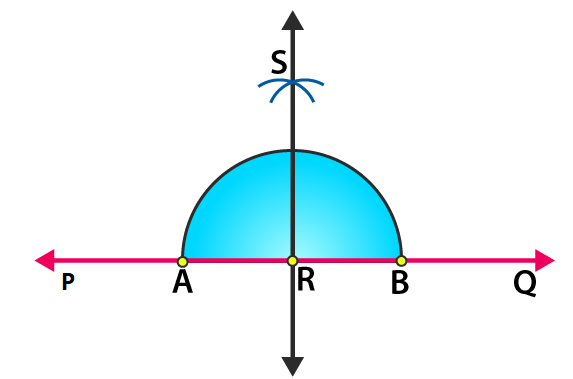3. Draw a line l. Take a point A, not lying on l. Draw a line m such that m ⊥ l and passing through A.

(Using (i) ruler and a set-square (ii) ruler and compasses)

Solution:

(i) Construct a line L and mark a point A outside it.

Now place the set square PQR with its one arm PQ of the right angle along the line L.

Place the ruler along the edge PR without disturbing the position of set square.

Slide the set square along the ruler until its arm QR reaches A without disturbing the position of the ruler.

Draw a line m.

Here, line m is the required line which is perpendicular to the line L.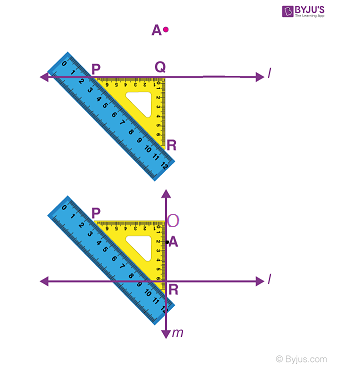(ii) Considering A as centre, construct an arc PQ which intersects the line L at P and Q.

By taking P and Q as centres, draw two arcs which intersects each other at the point B.

Now join the points A and B and extend in both the directions.

Here, AB is the required line.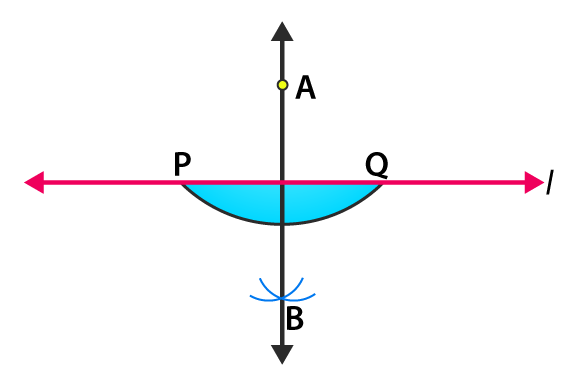4. Draw a line AB and take two points C and E on opposite sides of AB. Through C, draw CD ⊥ AB and through E draw EF ⊥ AB.

(Using (i) ruler and a set-square (ii) ruler and compasses)

Solution:

(i) Construct a line AB and mark two points C and E on the opposite sides of AB.

Place the set square PQR on the side E with its one arm PQ of the right angle along the line AB. Place the ruler along the edge PR.

Slide the set square along the ruler until the arm QR reaches C.

Construct a line CD where D is a point on the line AB.

Here CD is the required line where CD ⊥ AB

Now follow the same steps using a set square on the side E, we construct a line EF ⊥ AB.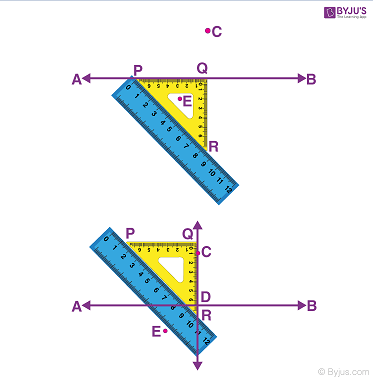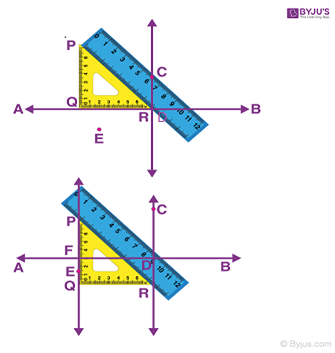(ii) Construct a line AB and mark two points C and E on the opposite sides.

Considering C as centre, construct an arc PQ which intersects AB at P and Q.

Considering P and Q as centres, draw two arcs which intersect each other at the point H.

Now join the points H and C where HC crosses the line AB at the point D.

We know that CD ⊥ AB

In the same way, consider E as centre and construct an arc RS.

Considering R and S as centres, construct two arcs which intersect each other at the point G.

Now join the point G and E such that GE crosses the line AB at the point F

We know that EF ⊥ AB.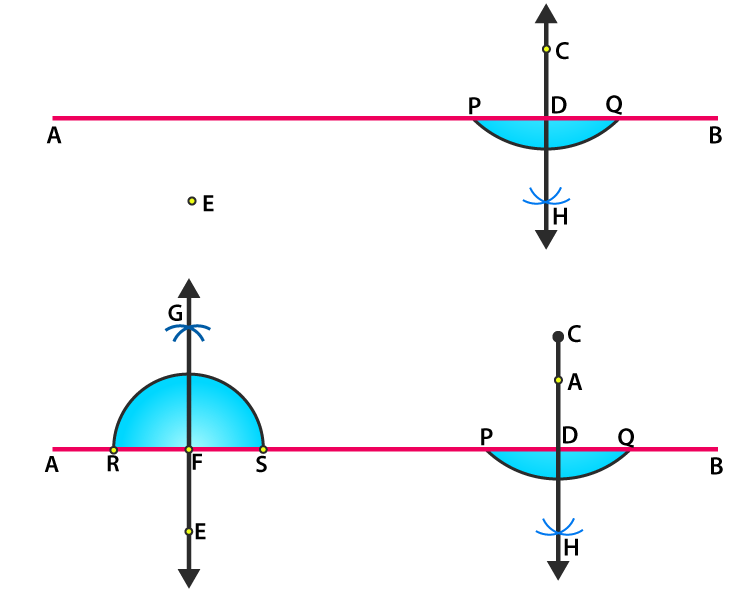5. Draw a line segment AB of length 10 cm. Mark a point P on AB such that AP = 4 cm. Draw a line through P perpendicular to AB.

Solution:

Construct a line L and mark a point A on it.

With the help of a ruler and a compass mark a point B which is at 10 cm from the point A on the line L.

Here, AB is the required line segment of length 10 cm.

Now, mark a point P which is 4 cm from the point A in the direction of B.

Taking P as centre and radius 4 cm draw an arc which intersects the line L at A and E.

Taking A and E as centres and radius 6 cm draw two arcs which intersects each other at the point R.

Now join P and R and extend it.

Here, PR is the required line which is perpendicular to the line AB.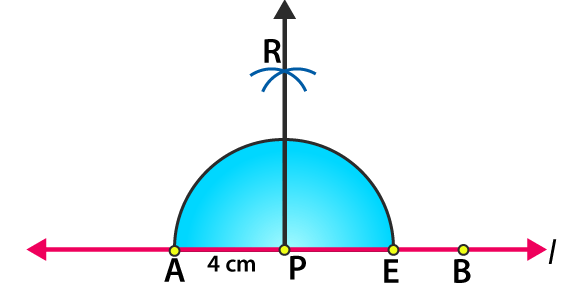6. Draw a line segment PQ of length 12 cm. Mark a point O outside this segment. Draw a line through O perpendicular to PQ.

Solution:

Construct a line L and mark a point P on it.

With the help of a ruler and a compass mark a point Q on the line L having a length PQ = 12 cm

Now mark a point O outside the line PQ.

Taking O as centre, construct an arc having appropriate radius such that the arc cuts the line at A and B.

Considering A and B as centres, draw two arcs such that they intersect each other at the point C.

Join the points OC.

Here, OC is the required line which is perpendicular to the line PQ.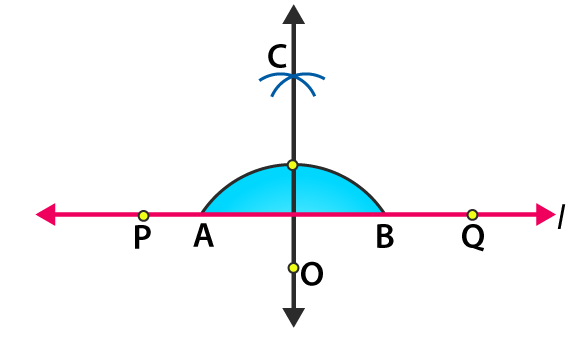7. Using a protractor, draw ∠BAC of measure 70o. On side AC, take a point P, such that AP = 2 cm. From P draw a line perpendicular to AB.

Solution:

Construct a line segment AC on the line L

(i) Place a protractor on the line segment AC such that it coincides with the line of diameter of protractor and the middle of the line coincides with A.

(ii) Now by counting from the right side, mark B as the point of 70o of the protractor and construct AB.

(iii) Measure 2 cm from the point A on the line segment AC and mark P.

(iv) Considering P as centre, construct an arc which intersects the line l at E and F.

(v) Considering the same radius and the points E and F as centres, draw two arcs which intersects G on the other side.

(vi) Join the points P and G.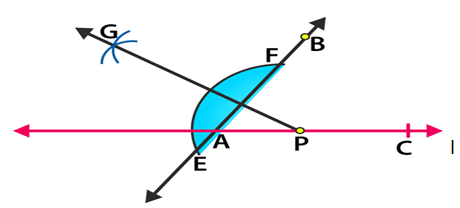8. Draw a line segment AB of length 8 cm. At each end of this line segment, draw a line perpendicular to AB. Are these two lines parallel?

Solution:

(i) By taking a convenient radius with A as centre construct an arc which intersects the line at W and X.

(ii) Taking W and X as centres and radius which is greater than AW, draw two arcs which intersects each other at the point M.

(iii) Now join the points AM and extend in both directions to P and Q.

(iv) With B as centre and convenient radius construct an arc which intersects the line at Y and Z.

(v) Considering Y and Z as centres and radius which is greater than YB, draw two arcs which intersects each other at the point N.

(vi) Now join the points BN and extend in both directions at the point S and R.

Consider the lines perpendicular to the points A and B be PQ and RS

We know that ∠QAB = ∠ABR = 90o

Two alternate interior angles are equal when the two parallel lines are intersected by a third line.

Hence, PQ and RS are parallel.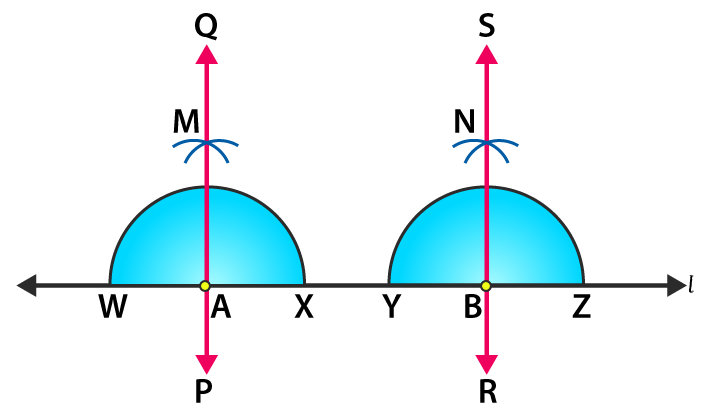9. Using a protractor, draw ∠BAC of measure 45o. Take a point P in the interior of ∠BAC. From P drawn line segments PM and PN such that PM ⊥ AB and PN ⊥ AC, Measure ∠MPN.

Solution:

(i) Construct a line segment A on the line L.

(ii) Place the protractor on the line segment AC such that it coincides with the line of the diameter of the protractor and the middle point of the line coincides with A.

(iii) By counting from the right side, mark B as the point of 45o of protractor and construct AB.

(iv) Taking P as centre and convenient radius draw an arc which intersects the line segment AB at points T and Q and AC at point R and S.

(v) Taking T and Q as centres and same radius, draw two arcs which intersects at point G on the other side.

(vi) Taking R and S as centres and same radius, draw two arcs which intersects at point H on the other side.

(vii) Now join the points PG and PH which intersects the line segments AB and AC at points M and N.

By measuring ∠MPN with the help of a protractor we get ∠MPN = 135o.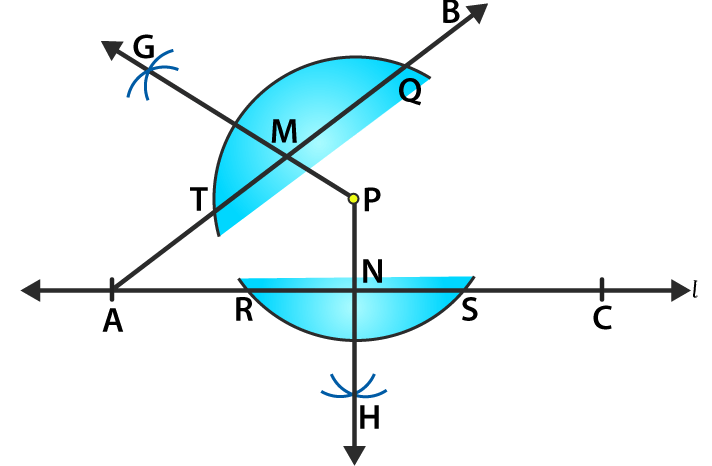10. Draw an angle and label it as ∠BAC. Draw its bisector ray AX and take a point P on it. From P draw line segments PM and PN, such that PM ⊥ AB and PN ⊥ AC, where M and N are respectively points on rays AB and AC. Measure PM and PN. Are the two lengths equal?

Solution:

(i) Construct ∠BAC on the line segment AC.

Considering A as centre with convenient radius, construct an arc from the line AB and AC.

(ii) Take both the points where the arc cuts the line segments AB and AC as centres, construct two small arcs which intersect at the point X and draw AX.

(iii) Now mark a point P on the ray AX.

(iv) Taking P as centre and convenient radius draw an arc which intersects AB at T and Q and AC at R and S.

(v) Taking T and Q as centres and same radius draw two arcs which intersects at point G on the other side.

(vi) Taking R and S as centres and same radius, draw two arcs which intersects at H on the other side.

(vii) Now join PG and PH which intersects the line segments AB and AC at the points M and N.

By measuring PM and PN with the help of a ruler, we get to know that both are equal.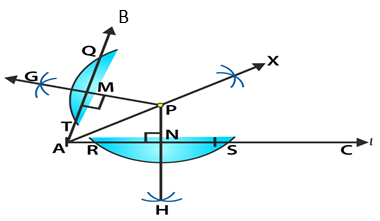### Exercise 19.3 page: 19.7

1. Draw a line segment of length 8.6 cm. Bisect it and measure the length of each part.

Solution:

Construct a line segment AB of length 8.6 cm.

Taking A as centre and radius which is more than half of line segment AB, construct arcs on both sides of AB.

Taking B as centre and same radius, construct arcs on both sides of AB which cuts the previous arcs at the points E and F.

Construct a line segment from the points E and F which intersects AB at the point C.

By measuring AC and BC we get AC = BC = 4.3 cm.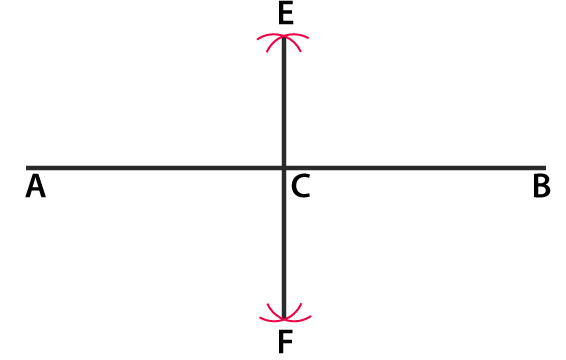2. Draw a line segment AB of length 5.8 cm. Draw the perpendicular bisector of this line segment.

Solution:

Construct a line segment AB of length 5.8 cm with the help of a ruler.

Taking A as centre and radius which is more than half of AB, construct arcs on both sides of the line segment AB.

Taking B as centre and same radius, construct arcs on both sides of AB which intersects the pervious arcs at the points L and M.

Construct the line segment LM with L and M as the end points.

Here, LM is the required perpendicular bisector of AB.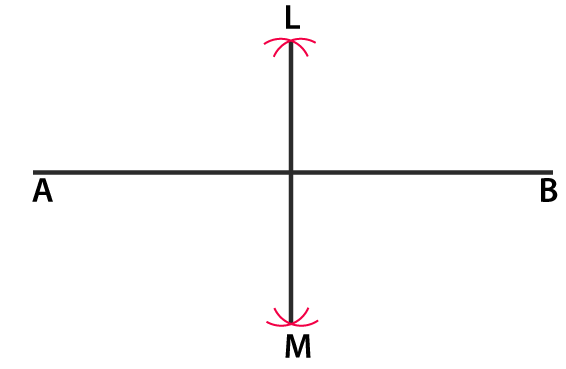3. Draw a circle with centre at point O and radius 5 cm. Draw its chord AB, draw the perpendicular bisector of line segment AB. Does it pass through the centre of the circle?

Solution:

Take a point O. By considering O as centre and radius which is equal to 5 cm construct a circle.

Now take points A and B on the circumference of the circle and construct a line segment with the points A and B as its end points.

Here AB is the chord of the circle.

Taking A as centre and radius which is more than half of AB, construct arcs on both sides of AB.

Taking B as centre and same radius, construct arcs on both sides of AB which cuts the previous arcs at the points E and F.

Construct a line passing through the points E and F.

Here, the line EF passes through the centre O of the circle.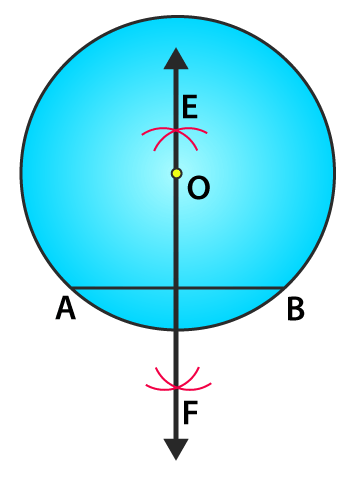4. Draw a circle with centre at point O. Draw its two chords AB and CD such that AB is not parallel to CD. Draw the perpendicular bisectors of AB and CD. At what point do they intersect?

Solution:

Construct a circle with O as the centre. Construct two chords AB and CD.

(i) Taking A as centre and radius more than half of AB construct arcs on both sides of the line segment AB.

(ii) Taking B as centre and same radius, construct arcs which cuts the previous arcs at the points P and Q.

(iii) Now join the points P and Q.

(iv) Taking C as centre and radius more than half of CD construct arcs on both sides of CD.

(v) Taking D as centre and same radius, construct arcs which cuts the previous arcs at the points R and S.

(vi) Now join the points R and S.

Construct the line segments of perpendicular bisector of AB and CD.

Here, the perpendicular bisector of line segments AB and CD meet at the point O which is the centre of the circle.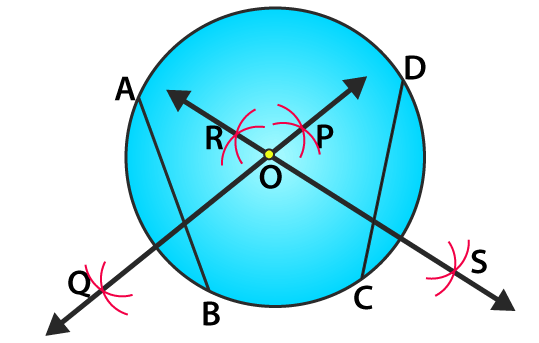5. Draw a line segment of length 10 cm and bisect it. Further bisect one of the equal parts and measure its length.

Solution:

Construct a line segment AB of length 10 cm and bisect it.

(i) Taking A as centre and radius which is more than half of AB, construct arcs on both sides of AB.

(ii) Taking B as centre and same radius, construct arcs which cuts the previous arcs at the points P and Q.

(iii) Now join the points P and Q which intersects the line AB at the point C.

(iv) Taking A as centre and radius which is more than half of AC, construct arcs on both sides of AB

(v) Taking C as centre and same radius, construct arcs which cuts the previous arcs at the points R and S.

(vi) Join the points R and S such that the line intersects AC at the point D.

Now by measuring AD with the help of a ruler we get AD = 2.5 cm.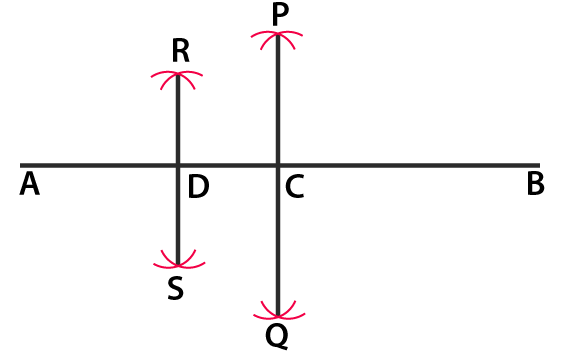6. Draw a line segment AB and bisect it. Bisect one of the equal parts to obtain a line segment of length 1/2 (AB).

Solution:

Construct a line segment AB.

(i) Taking A as centre and radius which is more than half of AB construct arcs on both sides of AB.

(ii) Taking B as centre and same radius, construct arcs which cuts the previous arcs at the points P and Q.

(iii) Now join the points P and Q such that the line PQ intersects AB at the point C.

(iv) Taking A as centre and radius more than half of AC construct arcs on both sides of AC.

(v) Taking C as centre and same radius construct arcs which cuts the previous arcs at the points R and S.

(vi) Now join the points R and S such that the line intersects AB at the point D.

Here, AC = CB = 1/2 (AB)

By dividing AC at D

We know that AD and AC are of same length

Hence, AD = AC = 1/4 (AB)7. Draw a line segment AB and by ruler and compasses, obtain a line segment of length 3/4 (AB).

Solution:

Construct a line segment AB with the help of a ruler.

(i) Taking A as centre and radius which is more than half of AB construct arcs on both sides of AB.

(ii) Taking B as centre and same radius construct arcs which cuts the previous arcs at the points P and Q.

(iii) Now join the points P and Q such that the line intersects AB at the point C.

(i) Taking A as centre and radius which is more than half of AB construct arcs on both sides of AC.

(ii) Taking C as centre and same radius construct arcs which cuts the previous arcs at the points R and S.

(iii) Now join the points R and S such that the line intersects AB at the point D.

Bisect AC and mark D as the point of bisection.

We know that

It can be written as

DC = 1/4 (AB) and CB = 1/2 (AB)

So we get

DB = 1/4 (AB) + 1/2 (AB) = 3/4 (AB)

Hence, DB is the required line segment of length 3/4 (AB).### Exercise 19.4 page: 19.9

1. Construct the following angles with the help of a protractor:

45o, 67o, 38o, 110o, 179o, 98o, 84o

Solution:

Construct a ray OA.

Now place the protractor on the ray OA such that its centre coincides with point O and the diameter coincides with OA.

Mark a point B against the mark of 45o on the protractor.

Remove the protractor and construct the line OB where ∠AOB is the required angle.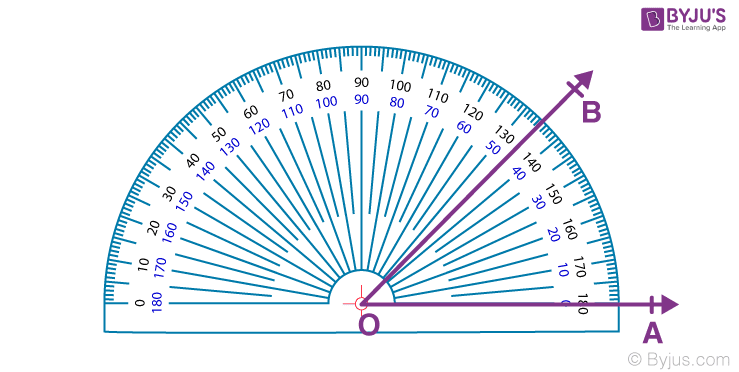In the same way draw the angles 67o, 38o, 110o, 179o, 98o and 84o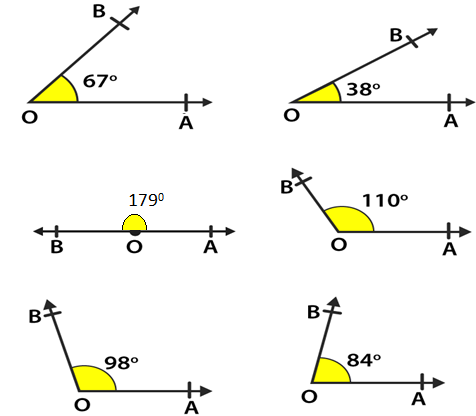2. Draw two rays PQ and RS as shown in Fig. 19.14 (i) and (ii). Using protractor, construct angles of 15o and 138o with one arm PQ and RS respectively.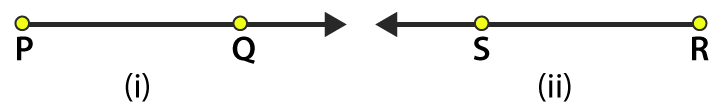Solution:

(i) Construct a ray PQ as given in the question.

Now place the protractor on PQ such that its centre coincides with the point P and the diameter coincides with PQ.

Mark B against the mark of 15o on the protractor.

Construct PB by removing the protractor.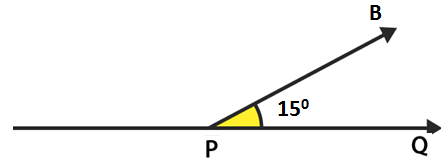Here ∠QPB is the required angle of 15o.

(ii) Construct a ray RS as given in the question.

Now place the protractor on RS such that its centre coincides with the point R and the diameter coincides with RS.

Mark T against the mark of 138o on the protractor.

Construct ST by removing the protractor.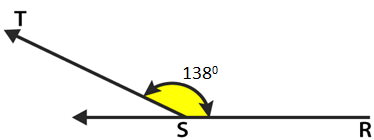Here ∠RST is the required angle of 138o.

### Exercise 19.5 page: 19.13

1. Draw an angle and label it as ∠BAC. Construct another angle, equal to ∠BAC.

Solution:

Construct an angle ∠BAC and draw a ray OP.

Taking A as centre and suitable radius, construct an arc which intersects AB and AC at points X and Y.

Taking O as centre and same radius, construct an arc which intersects the arc OP at the point M.

Now measure XY with the help of compass.

Taking M as centre and XY as radius construct an arc which intersects the arc which is drawn from O and name it as point N.

Now join the points O and N and extend it to the point Q.

Here, ∠POQ is the required angle.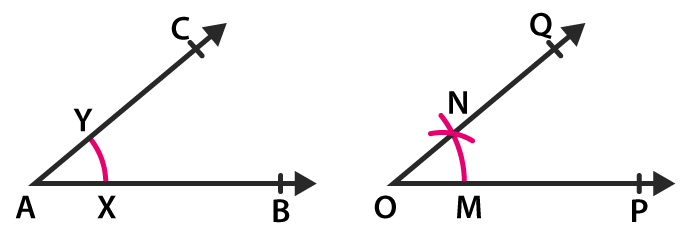2. Draw an obtuse angle. Bisect it. Measure each of the angles so obtained.

Solution:

We know that obtuse angles are those which are greater than 90o and less than 180o.

Construct an obtuse angle ∠BAC.

Taking A as centre with appropriate radius construct an arc which intersects AB and AC at the points P and Q.

Taking P as centre and radius which is more than half of PQ construct an arc.

Taking Q as centre and same radius construct another arc which intersects the previous arc at the point R.

Now join A and R and extend it to the point X.

So the ray AX is the required bisector of ∠BAC.

By measuring ∠BAR and ∠CAR we get ∠BAR = ∠CAR = 65o.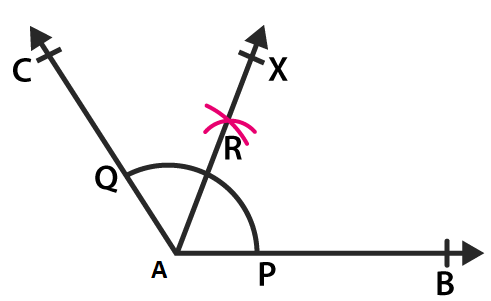3. Using your protractor, draw an angle of measure 108o. With this angle as given, drawn an angle of 54o.

Solution:

Construct a ray OA.

Using protractor, draw an angle ∠AOB of 108o where 108/2 = 54o

Hence, 54o is half of 108o.

In order to get angle 54o, we must bisect the angle of 108o.

Taking O as centre and convenient radius, construct an arc which cuts the sides OA and OB at the points P and Q.

Taking P as centre and radius which is more than half of PQ construct an arc.

Taking Q as centre and same radius construct another arc which intersects the previous arc and name it as point R.

Now join the points O and R and extend it to the point X.

Here, ∠AOX is the required angle of 54o.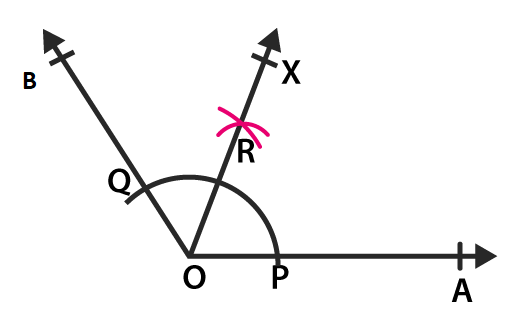4. Using protractor, draw a right angle. Bisect it to get an angle of measure 45o.

Solution:

Construct a ray OA.

Using a protractor construct ∠AOB of 90o.

Taking O as centre and convenient radius, construct an arc which cuts the sides OA and OB at the points P and Q.

Taking P as centre and radius which is more than half of PQ, construct an arc.

Taking Q as centre and same radius, construct another arc which intersects the previous arc and name it as point R.

Now join the points O and R and extend it to the point X.

Here, ∠AOX is the required angle of 45o where ∠AOB = 90o and ∠AOX = 45o.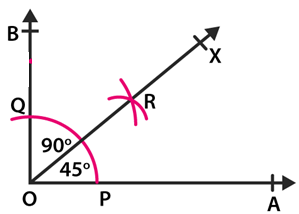5. Draw a linear pair of angles. Bisect each of the two angles. Verify that the two bisecting rays are perpendicular to each other.

Solution:

We know that the two angles which are adjacent and supplementary are known as linear pair of angles.

Construct a line AB and mark a point O on it.

By constructing an angle ∠AOC we get another angle ∠BOC.

Now bisect ∠AOC using a compass and a ruler and get the ray OX.

In the same way bisect ∠BOC and get the ray OY.

We know that

∠XOY = ∠XOC + ∠COY

It can be written as

∠XOY = 1/2 ∠AOC + 1/2 ∠BOC

So we get

∠XOY = 1/2 (∠AOC + ∠BOC)

We know that ∠AOC and ∠BOC are supplementary angles

∠XOY = 1/2 (180) = 90o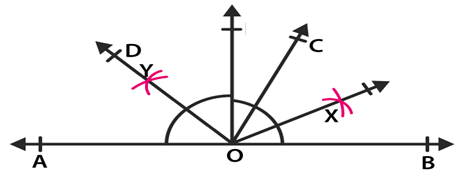6. Draw a pair of vertically opposite angles. Bisect each of the two angles. Verify that the bisecting rays are in the same line.

Solution:

Construct two lines AB and CD which intersects each other at the point O

Since vertically opposite angles are equal we get

∠BOC = ∠AOD and ∠AOC = ∠BOD

Now bisect angle AOC and construct the bisecting ray as OX.

In the same way, we bisect ∠BOD and construct bisecting ray OY.

We get

∠XOA + ∠AOD + ∠DOY = 1/2 ∠AOC + ∠AOD + 1/2 ∠BOD

We know that ∠AOC = ∠BOD

∠XOA + ∠AOD + ∠DOY = 1/2 ∠BOD + ∠AOD + 1/2 ∠BOD

So we get

∠XOA + ∠AOD + ∠DOY = ∠AOD + ∠BOD

AB is a line

We know that ∠AOD and ∠BOD are supplementary angles whose sum is equal to 180o.

∠XOA + ∠AOD + ∠DOY = 180o

The angles on one side of a straight line is always 180o and also the sum of angles is 180o

Here, XY is a straight line where OX and OY are in the same line.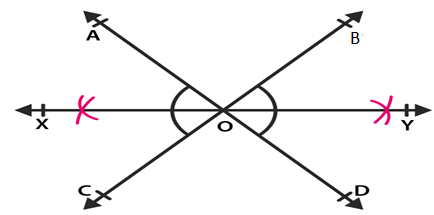7. Using ruler and compasses only, draw a right angle.

Solution:

Construct a ray OA.

Taking O as centre and convenient radius construct an arc PQ using a compass intersecting the ray OA at the point Q.

Taking P as centre and same radius construct another arc which intersects the arc PQ at the point R.

Taking R as centre and same radius, construct an arc which cuts the arc PQ at the point C opposite to P.

Using C and R as the centre construct two arcs of radius which is more than half of CR intersecting each other at the point S.

Now join the points O and S and extend it to the point B.

Here, ∠AOB is the required angle of 90o.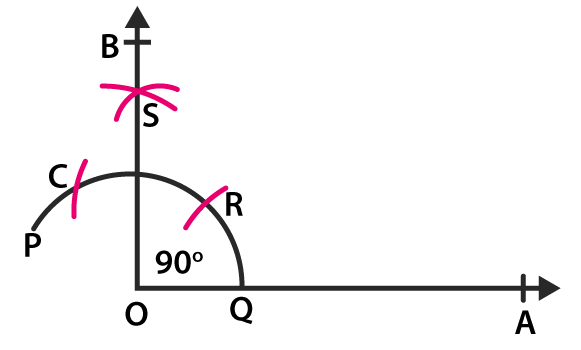8. Using ruler and compasses only, draw an angle of measure 135o.

Solution:

Construct a line AB and mark a point O on it.

Taking O as centre and convenient radius, construct an arc PQ using a compass which intersects the line AB at the point P and Q.

Taking P as centre and same radius, construct another arc which intersects the arc PQ at the point R.

Taking Q as centre and same radius, construct another arc which intersects the arc PQ at the point S which is opposite to P.

Considering S and R as centres and radius which is more than half of SR, construct two arcs which intersects each other at the point T.

Now join the points O and T which intersects the arc PQ at the point C.

Considering C and Q as centres and radius which is more than half of CQ, construct two arcs which intersects each other at the point D.

Now join the points O and D and extend it to point X to form the ray OX.

Here, ∠AOX is the required angle of 135o.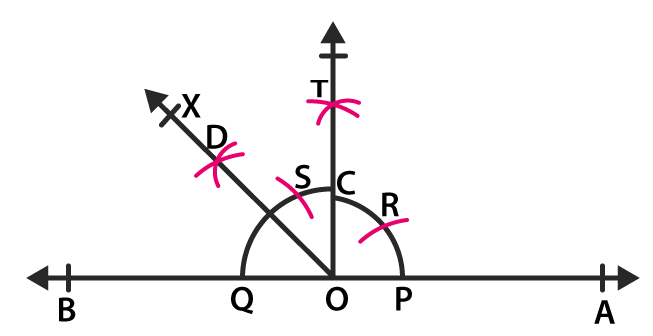9. Using a protractor, draw an angle of measure 72o. With this angle as given, draw angles of measure 36o and 54o.

Solution:

Construct a ray OA.

Using protractor construct ∠AOB of 72o

Taking O as centre and convenient radius, construct an arc which cut sides OA and OB at the point P and Q.

Taking P and Q as centres and radius which is more than half of PQ, construct two arcs which cuts each other at the point R.

Now join the points O and R and extend it to the point X.

Here, OR intersects the arc PQ at the point C.

Taking C and Q as centres and radius which is more than half of CQ, construct two arcs which cuts each other at point T.

Now join the points O and T and extend it to the point Y.

OX bisects ∠AOB

It can be written as

∠AOX = ∠BOX = 72/2 = 36o

OY bisects ∠BOX

It can be written as

∠XOY = ∠BOY = 36/2 = 18o

We know that

∠AOY = ∠AOX + ∠XOY = 36o + 18o = 54o

Here, ∠AOX is the required angle of 36o and ∠AOY is the required angle of 54o.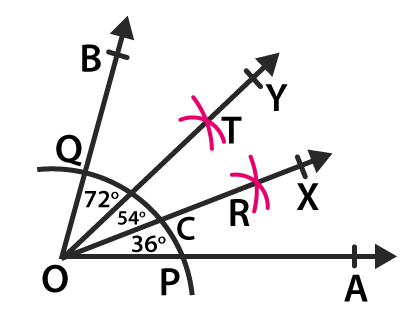### Exercise 19.6 page: 19.15

1. Construct an angle of 60o with the help of compasses and bisect it by paper folding.

Solution:

Construct a ray OA

Taking O as centre and convenient radius, construct an arc which cuts the ray OA at the point P.

Taking P as centre and same radius, construct another arc which cuts the previous arc at the point Q.

Construct OQ and extend it to the point B.

Here, ∠AOB is the required angle of 60o

Now cut the part of paper as sector OPQ

Fold the part of paper such that the line segments OP and OQ coincides.

The angle which is made at point O is the required angle which is half of ∠AOB.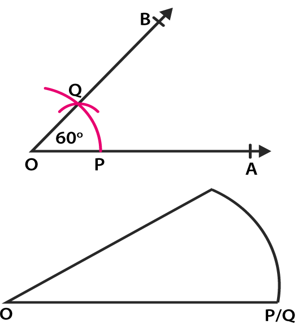2. Construct the following angles with the help of ruler and compasses only:

(i) 30o

(ii) 90o

(iii) 45o

(iv) 135o

(v) 150o

(vi) 105o

Solution:

(i) 30o

Construct a ray OA.

Taking O as centre and with convenient radius, construct an arc which cuts OA at the point P.

Taking P as centre and same radius, construct an arc which cuts the previous arc at the point Q.

Considering P and Q as centres and radius which is more than half of PQ construct two arcs which cuts each other and name it as point R.

Construct OR and extend it to the point B.

Here, ∠AOB is the required angle of 30o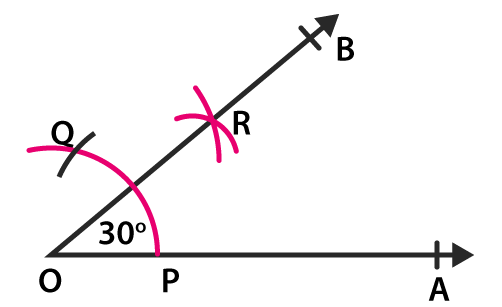(ii) 90o

Construct a ray OA.

Taking O as centre and with convenient radius, construct an arc which cuts OA at the point P.

Taking P as centre and same radius, construct an arc which cuts the previous arc at the point Q.

Taking Q as centre and same radius, construct an arc which cuts the previous arc at the point R.

Considering Q and R as centres and radius which is more than half of QR construct two arcs which cuts each other and name it as point S.

Construct OS and extend it to the point B from the ray OB.

Here, ∠AOB is the required angle of 90o.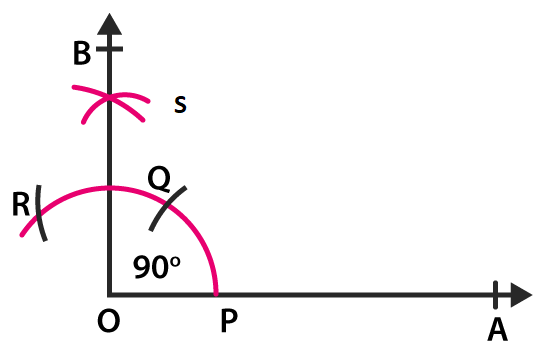(iii) 45o

In order to draw an angle of 45o, construct an angle of 90o and bisect it.

Construct ∠AOB = 90o where OA and OB are the rays which intersect the arc at the points P and T

Taking P and T as centres and radius which is more than half of PT, construct two arcs which cuts each other and name it as point X

Construct OX and extend it to the point C to form the ray OC

Here, ∠AOC is the required angle of 45o.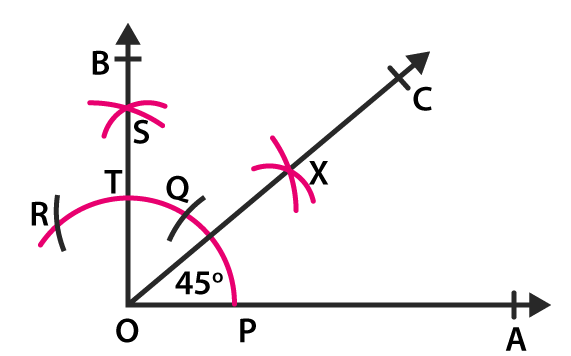(iv) 135o

Construct a line AB and mark a point O in the middle of AB.

Taking O as centre and convenient radius, construct an arc which cuts the line AB at the points P and Q.

Construct an angle of 90o on the ray OB as ∠BOC = 90o where OC cuts the arc at the point R

Taking Q and R as centres and radius which is more than half of QR, construct two arcs which cuts each other and name it as point S.

Construct OS and extend it to the point D to form the ray OD.

Here, ∠BOD is the required angle of 135o.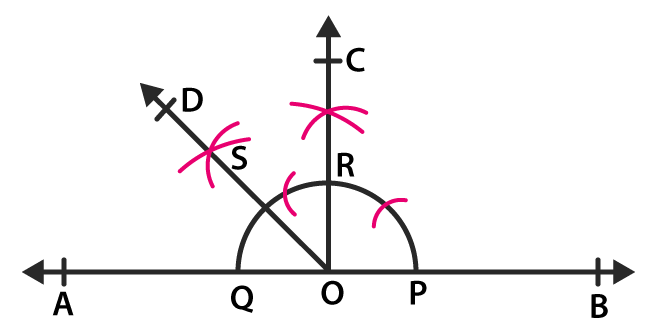(v) 150o

Construct a line AB and mark a point O in the middle of AB.

Taking O as centre and convenient radius construct an arc which cuts the line AB at the points P and Q.

Taking Q as centre and same radius construct an arc which cuts the previous arc and name it as point R.

Taking R as centre and same radius construct an arc which cuts the previous arc and name it as point S.

Taking P and S as centres and radius which is more than half of PS, construct two arcs which cuts each other and name it as point T.

Construct OT and extend it to the point C to form the ray OC.

Here, ∠BOC is the required angle of 150o.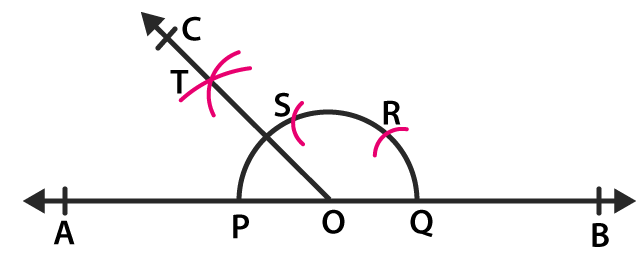(vi) 105o

Construct a ray OA and make ∠AOB = 90o and ∠AOC = 120o

Bisect ∠BOC and get the ray OD.

Here, ∠AOD is the required angle of 105o.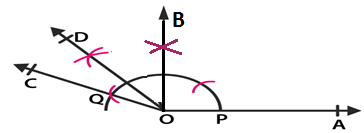3. Construct a rectangle whose adjacent sides are 8 cm and 3 cm.

Solution:

Construct a line segment AB of length 8 cm.

Draw ∠BAX = 90o at A and ∠ABY = 90o at B

With the help of compass and ruler, mark a point D on the ray AX where AD = 3 cm

In the same way mark the point C on the ray BY where BC = 3 cm

Construct the line segment CD

Hence, ABCD is the required rectangle.